# JP3793115B2 - 3D computer modeling device - Google Patents

## Info

Publication number
JP3793115B2
JP3793115B2 JP2002169584A JP2002169584A JP3793115B2 JP 3793115 B2 JP3793115 B2 JP 3793115B2 JP 2002169584 A JP2002169584 A JP 2002169584A JP 2002169584 A JP2002169584 A JP 2002169584A JP 3793115 B2 JP3793115 B2 JP 3793115B2
Authority
JP
Japan
Prior art keywords
volume
plurality
step
polyhedron
3d
Prior art date
Legal status (The legal status is an assumption and is not a legal conclusion. Google has not performed a legal analysis and makes no representation as to the accuracy of the status listed.)
Expired - Fee Related
Application number
JP2002169584A
Other languages
Japanese (ja)
Other versions
JP2003216979A (en
Inventor
ウィリアム クリストファー コッチェフ アーロン
マイケル ボームバーグ アダム
ラルフ リオンズ アレキサンダー
マイケル ロウ サイモン
Original Assignee
キヤノン株式会社
Priority date (The priority date is an assumption and is not a legal conclusion. Google has not performed a legal analysis and makes no representation as to the accuracy of the date listed.)
Filing date
Publication date
Priority to GB0114157A priority Critical patent/GB0114157D0/en
Priority to GB0114157.1 priority
Application filed by キヤノン株式会社 filed Critical キヤノン株式会社
Publication of JP2003216979A publication Critical patent/JP2003216979A/en
Application granted granted Critical
Publication of JP3793115B2 publication Critical patent/JP3793115B2/en
Expired - Fee Related legal-status Critical Current
Anticipated expiration legal-status Critical

• 238000005094 computer simulation Methods 0.000 title claims description 80
• 238000000034 methods Methods 0.000 claims description 187
• 238000007689 inspection Methods 0.000 claims description 30
• 238000004364 calculation methods Methods 0.000 claims description 25
• 230000000875 corresponding Effects 0.000 claims description 9
• 230000001808 coupling Effects 0.000 claims description 2
• 238000010168 coupling process Methods 0.000 claims description 2
• 238000005859 coupling reactions Methods 0.000 claims description 2
• 239000000203 mixtures Substances 0.000 description 8
• 230000000694 effects Effects 0.000 description 5
• 239000000463 materials Substances 0.000 description 3
• 238000005516 engineering processes Methods 0.000 description 2
• 238000006243 chemical reactions Methods 0.000 description 1
• 238000004590 computer program Methods 0.000 description 1
• 238000010276 construction Methods 0.000 description 1
• 230000001276 controlling effects Effects 0.000 description 1
• 230000004044 response Effects 0.000 description 1

## Images

•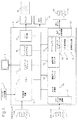•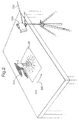•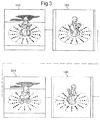•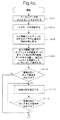•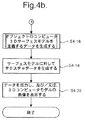•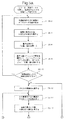•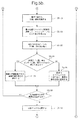•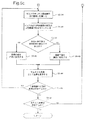•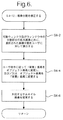•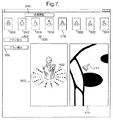•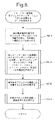•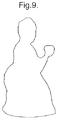•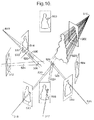•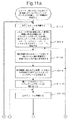•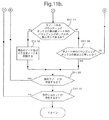•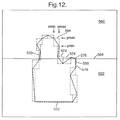••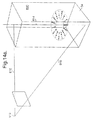••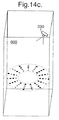•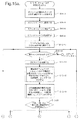•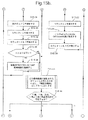•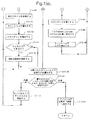•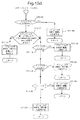•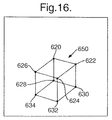••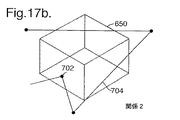•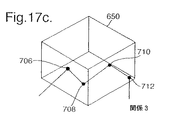•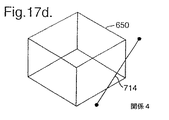•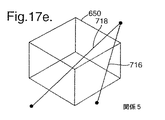••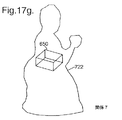•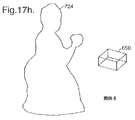•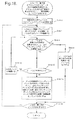•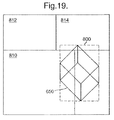•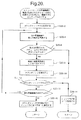•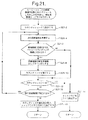•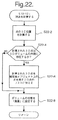•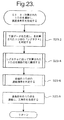•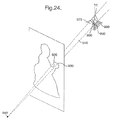•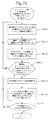•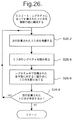•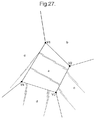•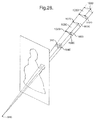••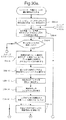•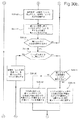•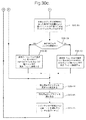•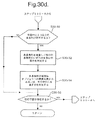•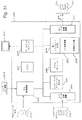•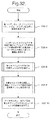•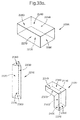•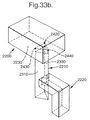•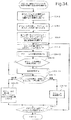•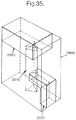••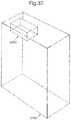•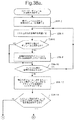•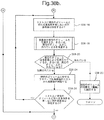•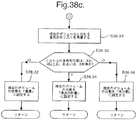•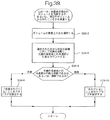•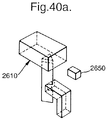•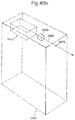•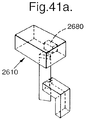•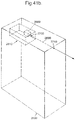•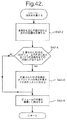•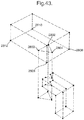•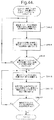•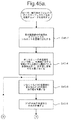•## Classifications

• GPHYSICS
• G06COMPUTING; CALCULATING; COUNTING
• G06TIMAGE DATA PROCESSING OR GENERATION, IN GENERAL
• G06T17/00Three dimensional [3D] modelling, e.g. data description of 3D objects
• G06T17/20Finite element generation, e.g. wire-frame surface description, tesselation

## Description


The present invention relates to computer processing for generating a three-dimensional (3D) computer model of an object. In particular, the present invention relates to a computer process for generating a 3D computer model by calculating a common part of a polyhedron (pplyhedra) each defining a volume in 3D space containing at least a portion of an object.

Many methods are known as methods for generating a 3D computer model of an object.

A known method is the “shape-from-silhouette” method. This method processes the image of the object recorded at a known position and orientation, backprojects the silhouette of the object in each image, and includes the object and the vertex at the focal position of the camera when the image was recorded. Generate a 3D computer model by creating an infinite cone. Thus, by constraining the volume of 3D space that each cone occupies, the intersection of each cone that approximates the object is calculated.

Examples of shape-from-silhouette methods are, for example, Illingworth and Hilton “Looking to build a model world: automatic construction of static object models using computer vision” (Electronics and Communication Engineering Journal, June 1998, pages 103-113). And Niem's “Automatic reconstruction of 3D objects using a mobile camera” (Image and Vision Computing 17, 1999, pages 125-134). However, in the methods described in both of these papers, the intersection of silhouette cones is calculated to generate a “volume representation” of an object composed of a plurality of voxels (straight parallelepipeds). Specifically, the 3D space is divided into a plurality of voxels and these voxels are examined to determine which voxels are located inside the volume defined by the intersection of silhouette cones. Voxels inside the intersection volume are retained to define a plurality of voxel volumes representing the object.

However, such voxel technology has several problems. In particular, the accuracy and resolution of the resulting volume representation of the object is determined by the dimensions of each voxel into which the 3D space is divided. For this reason, when the model resolution is improved by reducing the dimensions of the voxels, the number of voxels that need to be inspected increases significantly, resulting in a longer processing time. In addition, most computer graphics rendering algorithms cannot render a voxel model and generate an image for display to the user, so a voxel representation is a surface model consisting of multiple polygons. Needs to be converted to This conversion not only requires additional processing resources and processing time, but may reduce the resolution and accuracy of the resulting computer model.

Martin Lohlein's “A Volumetric Intersection Algorithm for 3d-Reconstruction Using a Boundary-Representation” (http: // i31www.ira.uka.de/diplomarbeiten/da_martin_loehlein/Reconstruction.html) shape-from that generates a 3D computer model The -silhouette method is disclosed, but this method does not generate a voxel representation. Instead, the intersection of each silhouette cone based on multiple images is calculated directly. Specifically, the method first prepares a cube containing the object and intersects the first silhouette cone to obtain a first approximation of the object. Subsequently, this approximation is intersected with the next cone, and the same processing is repeated. In order to intersect the silhouette cone with the approximation, the cone and approximation are projected onto the image from which the cone was created. This returns the cone to the original 2D polygon (silhouette) and the approximation from the 3D polygon back to the 2D polygon. The conical polygon is directed to intersect all approximate polygons using a conventional algorithm for intersection of 2D polygons.

However, the method described in “A Volumetric Intersection Algorithm for 3d-Reconstruction Using a Boundary-Representation” is inefficient in terms of processing resources and processing time. One reason for this problem is that the inventors have generated a more accurate representation consisting of a plurality of connected 3D points that define a plane by intersecting a silhouette cone with the representation, and this representation. It is recognized that this is an incremental method that intersects with another silhouette cone and repeats the same process.

M. Szilvasi-Nagy's “An Algorithm for Determining the Intersection of Two Simple Polyhedra” (Computer Graphics Forum 3, 1984, pp. 219-225) uses the “plane-sweep algorithm” to identify the edges of each polyhedron. A method is disclosed for computing the intersection of two simple polyhedrons, such as a tetrahedron and a prism, by finding all intersections with the faces of one polyhedron. This method is useful for two polyhedrons each having a small number of faces, but in the case of three or more polyhedra and / or a polyhedron having a large number of faces, from the viewpoint of computational resources and computation time. Would be extremely inefficient.

The present invention has been made in view of the above-described problems, and its object is to address one or more of the problems.

In accordance with the present invention, there is provided a computer processing method and apparatus for generating a non-voxel representation of an object by intersecting at least three polyhedra. Each point of the common part of each plane that constitutes the polyhedron is calculated / inspected to determine which point corresponds to the point of the object. Each object point is labeled with a plane that intersects there. Thereafter, when the identification of all the object points is completed, the connection between the object points is specified according to the label.

As described above, when all of the object points are calculated before determining the connection between the object points, the processing for determining the connection need only be executed once, so that processing resources and / or processing time are inevitably saved. Is done. This is made possible by labeling each object point based on the plane that intersects to produce each object point, and calculating which object points should be connected to each other using these labels .

The present invention also provides a computer processing method and apparatus for generating a non-voxel representation of an object by intersecting a plurality of polyhedra. The initial 3D volume with the object inside is divided into a plurality of parts, and each part is inspected against a polyhedron. If a portion of the volume is outside the at least one polyhedron, it is discarded because it cannot contain a point on the surface of the object. If not outside the polyhedron, the face of the polyhedron that intersects the volume is processed and each point of the common part is calculated. Therefore, a 3D computer model composed of the calculated points of the common part is generated.

In this way, since the calculation of the common part that cannot represent the point on the object is avoided, it is not necessary to calculate all the common parts of the faces of the polyhedron, leading to a reduction in processing resources and processing time.

The process is performed more efficiently by iteratively repartitioning the initial volume and calculating the intersection of the polyhedral faces for that part only if a portion of the volume intersects a few polyhedral faces. Is preferred. To illustrate, in one embodiment, each common portion comprises a common portion of three polyhedral faces. Therefore, it is necessary to inspect each combination of three surfaces that intersect a part of the volume and determine whether or not they intersect each other. As a result, when a part of the volume intersects with many polyhedron faces, a considerable number of calculations are performed. Since the points in the common part do not represent points on the object, much of the computation is wasted. However, by repartitioning the initial volume repeatedly, a large volume can be discarded without having to calculate the intersection, and only when a sufficiently small part of the volume intersecting a few polyhedron faces is obtained. By calculating the common part, the number of common part calculations that must actually be performed is minimized.

The present invention may also be embodied as a storage device or signal including instructions for configuring a programmable processing device to be operable to perform the above-described method or configured as the above-described device. Computerized computer program product is provided.

Embodiments of the present invention will be described by way of example only with reference to the accompanying drawings.

First embodiment
Referring to FIG. 1, an embodiment of the present invention is a conventional personal computer including a processing device 2 such as a personal computer including one or more processors, a plurality of memories, a graphics card, and the like. A display device 4 such as a monitor, a user input device 6 such as a keyboard and a mouse, a printer 8, and a display panel 10 composed of a flat panel having controllable pixels such as PL400 manufactured by WACOM. .

The processing device 2 is input to the processing device 2 as data stored in a data storage medium such as the disk 12 and / or from a remote database or the like via a communication network (not shown) such as the Internet, or by wireless transmission. And / or programmed to operate according to programming instructions entered as a signal 14 entered by the user via a user input device 6 such as a keyboard.

As described in more detail below, these programming instructions are used to calculate one or more subject objects recorded at various different positions and orientations to calculate the position and orientation at which the input image was recorded. Instructions for configuring the processing device 2 to process input data defining a plurality of images and generate data defining a three-dimensional computer model of a subject object using the calculated position and orientation is there. In this embodiment, the subject object is photographed on a calibration object (a two-dimensional photographic mat in this embodiment) having a known feature pattern. The position and orientation when the input image is recorded are calculated by detecting the position of the feature of the calibration object pattern in the image. A 3D computer model of the subject object is generated using a novel inventive polyhedra intersection technology. In this technique, the contour of the subject object in each image is divided into a plurality of portions, and 3D points representing each point on the surface of the subject object are determined by determining the common part of the polyhedron defined by the 3D projection of the contour portion. Processing for calculation is executed. As will be described in more detail below, this process is particularly efficient because it is not necessary to calculate the common part of each face in the polyhedron that cannot generate 3D points on the surface of the subject object. It is executed in a way.

The processing device 2 can be considered as being configured as several functional units for performing processing operations when programmed with programming instructions. Examples of such functional units and their interconnection relationships are shown in FIG. However, the units and interconnections shown in FIG. 1 are conceptual and are shown by way of example only for the purpose of facilitating understanding. Therefore, they do not necessarily represent units or connections in which the processor, memory, etc. of the processing device 2 are configured.

Referring to the functional unit shown in FIG. 1, the central control device 20 is configured to process the input from the user input device 6 and to control and process other functional units. Memory 24 is provided for use by central controller 20 and other functional units.

The mat generator 30 prints a calibration pattern on a recording medium such as paper to form a printed photo mat, or displays the calibration pattern on the display panel 10 to display the photo mat. A control signal for controlling the printer 8 or the display panel 10 is generated. As will be described in more detail below, the photo mat has a calibration pattern of predetermined characteristics, and the object for which a three-dimensional computer model is to be generated is a printed photo mat 34 or a display panel on which the calibration pattern is displayed. 10 is arranged. Thereafter, the image of the object and the calibration pattern are recorded and input to the processing device 2. The mat generator 30 stores data defining a calibration pattern of features printed or displayed on a photo mat for use by the processing device 2 in calculating the position and orientation during recording of the input image. Composed. That is, in this embodiment, the mat generator 30 is configured to store the data defining the feature pattern together with the coordinate system for the feature pattern (actually defining the reference position and orientation of the calibration pattern). The processing device 2 is configured to calculate the position and orientation when the input image is recorded in a predefined coordinate system (and thus relative to the reference position and reference orientation).

In this embodiment, the calibration pattern of the photographic mat is a spatial cluster of features as described, for example, in PCT patent application GB00 / 04469, the contents of which are all incorporated herein by reference, or A known pattern of colored dots, such as described in JP-A-9-170914, where each dot has a different hue / brightness combination such that each corresponding dot is unique, e.g. Niem's “Automatic Reconstruction of 3D Objects Using A Mobile Camera” (Image and Vision Computing 17 (1999), pages 125-134), dimensions are known for multiple concentric circles in each quadrant Patterns formed by connecting radial segments and position markers, or Gortler et al. “The Lumigraph” (Computer Graphics Proceedings, A nnual Conference Series, 1996, ACM-0-89791-764-4 / 96/008, and a pattern composed of a plurality of concentric rings each having a different diameter.

In the following description of this embodiment, it is assumed that the calibration pattern is printed on a recording medium (sheet paper in this embodiment) by the printer 8 in order to generate the printed photo mat 34. As described above, the calibration pattern may be displayed on the display panel 10.

The input data storage device 40 is input to the processing device 2 as data stored in a storage device such as a disk 42 or as a signal 44 transmitted to the processing device 2 or using the user input device 6. It is configured to store input data. The input data indicates a plurality of images of one or more subject objects on a photo mat recorded at various different positions and orientations, and different objects having the same color as the background color of the photo mat or the background color of the photo mat An input image showing a background on which an object is photographed is defined together with a part of a photo mat for the purpose. In addition, in this embodiment, the input data includes parameters specific to the camera that recorded the image, that is, aspect ratio, focal length, principal point (point where the optical axis intersects the imaging plane), primary radial distortion coefficient, and skew angle. Also included is data defining (the angle formed by the axes of the pixel grid, since the axes of the pixel grid may not be strictly orthogonal).

The input data defining the input image may be generated, for example, by downloading pixel data from a digital camera that recorded the image or by scanning a photograph using a scanner (not shown). Input data defining the unique camera parameters may be input from the user side using the user input device 6.

The camera calculator 50 processes each input image to detect the position of the calibration pattern feature on the photographic mat in the image and to calculate the position and orientation of the camera when the input image is recorded. Composed.

The image data segmenter 60 is configured to process each input image and separate image data corresponding to the subject object from other image data in the image.

The image segmentation editor 70 is operable to edit the segmented image data generated by the image data segmenter 60 under the control of the user. As will be described in more detail below, this allows the user to correct the image segmentation generated by the image data segmenter 60, in particular, for example, to correct pixels incorrectly determined by the image data segmenter 60. Can be associated with the subject object 210 (eg, a photo mat 34 and / or pixels associated with a mark or other feature visible on the surface on which the subject object is placed for shooting). All of the pixels associated with shading on the image plane and the pixels associated with the calibration features on the photo mat 34 that touch the contour of the subject object in the input image are misclassified and not corrected during image data segmentation The resulting 3D computer model may be inaccurate).

A surface modeler (surface modeler) 80 is divided image data generated by the image data segmenter 60 and the image dividing editor 70, and data defining the position and orientation when the image generated by the camera calculator 50 is recorded. Are processed to generate data defining a 3D computer model that represents the actual surface of the object in the input image.

The surface modeler 80 includes a silhouette axiometer 82, a 3D point calculator 84, and a polygon generator 86.

A silhouette approximator 82 processes segmented image data generated for each input image by the image data segmenter 60 and the image segmentation editor 70, and approximates the silhouette of the subject object in each image by line segments. Configured to do.

The 3D point calculator 84 is configured to process the line segment generated by the silhouette axiometer 82 and calculate the intersection of the polygons defined by the projection of the line segment into a three-dimensional space. These intersections define 3D points that potentially exist on the surface of the subject object. The 3D point calculator 84 is configured to examine the calculated 3D points to determine which points represent actual points in the subject object.

A polygon generator 86 is configured to connect the 3D points generated by the 3D point calculator 84 to form a polygon mesh representing the surface of the subject object.

Specifically, since the common part of the polygon calculated by the 3D point calculator 84 defines the visual body of the subject object, these 3D points are included in the polygon mesh representing the surface of the subject object. Are connected by a polygon generator 86 to form the vertices of the polygons. For this reason, the 3D points calculated by the 3D point calculator 84 are hereinafter referred to as 3D vertices.

Surface texturer 90 is configured to generate texture data from input image data for rendering to a surface model generated by surface modeler 80.

The display processor 110 is configured to display images and instructions to the user via the display device 4 during the process of generating the 3D computer model under the control of the central controller 20. Further, under the control of the central controller 20, the display processor 110 processes the surface model data generated by the surface modeler 80 and renders the texture data generated by the surface texturer 90 on the surface model. The 3D computer model image of the object is displayed from the viewpoint selected by the user.

The output data store 120 is configured to optionally store texture data generated by the surface texturer 90 in addition to storing data defining the surface model generated by the surface modeler 80. The central controller 20 controls the output of data from the output data storage device 120 as data and / or signals 124 to a storage device such as the disk 122, for example.

Referring to FIG. 2, a printed photo mat 34 is placed on the surface 200, and a subject object 210 for which a 3D computer model is to be generated is surrounded by features that make up the calibration pattern on the mat. Is disposed on the photo mat 34.

The surface 200 preferably has a substantially uniform color, and if possible, during the division processing by the image data segmenter 60, the image data related to the subject object 210 is accurately extracted from other image data in the input image. It is made different from any color in the subject object 210 so that it can be distinguished. However, if it is the same as one of the colors, for example, if a mark 220 having the same color as the color in the subject object 210 appears on the surface 200 (and thus also appears in the input image), this embodiment Then, as will be described later in detail, by allowing the user to edit the divided data generated by the image data segmenter 60, processing can be executed to realize this.

To show different parts of the subject object 210, the subject object 210 and the image of the photographic mat 34 are recorded at different positions and orientations using the digital camera 230. In this embodiment, data defining an image recorded by the camera 230 is input to the processing device 2 as a signal 44 via the wire 232.

More specifically, in this embodiment, the position of the camera 230 remains fixed, and the photographic mat 34 carrying the subject object 210 moves (translates) on the surface 200 (for example, in the direction of the arrow 240). The photograph of the subject object 210 is recorded at different positions and orientations relative to the camera 230. During rotation and translation of the photo mat 34 on the surface 200, the subject object 210 does not move relative to the mat 34.

FIG. 3 shows examples of images 300, 302, 304, and 306 that are defined in the data input to the processing device 2 of the subject object 210 and the photographic mat 34 that are in various different positions and orientations relative to the camera 230.

In this embodiment, following the recording / input of the image of the subject object 210 and the photographic mat 34, another image is recorded and input to the processing device 2. This other image is a “background image” that is an image of the surface 200 and an object having the same color as the paper on which the photo mat 34 is printed. Such a background image has a sheet of unprinted paper of the same color as the paper on which the photographic mat 34 is recorded placed on the surface 200 or the pattern is not visible in the image. Alternatively, recording may be performed by turning the photo mat 34 over and placing it on the surface 200.

FIG. 4 shows the processing operations performed by the processing device 2 to process input data in this embodiment.

In step S4-2 in FIG. 4, the central control device 20 causes the display device 4 to display a message requesting the display processor 110 to input input data to be processed by the user.

In step S4-4, the data input by the user in response to the request in step S4-2 is stored in the input data storage device 40. That is, in this embodiment, the input data includes image data defining images of the subject object 210 and the photo mat 34 recorded at various different positions and orientations with respect to the camera 230, and a photograph for recording the input image. A “background image” indicating both the surface 200 on which the mat 34 is arranged and an object having the same color as the recording material on which the calibration pattern of the photographic mat 34 is printed, and a specific parameter of the camera 230 that records the input image; That is, it is composed of data defining the aspect ratio, focal length, principal point (point where the optical axis intersects the imaging plane), primary radial distortion coefficient, and skew angle (angle formed by the pixel grid axis).

In step S4-6, the camera calculator 50 processes the input data stored in step S4-4, and for each input image, the camera 230 performs the calibration pattern on the photographic mat 34 (and thus the subject object 210). Determine position and orientation. This process detects, for each input image, features in the image constituting the calibration pattern of the photo mat 34 and compares those features with the stored photo mat pattern to compare the features of the camera 230 to the mat. It consists of determining the position and orientation. The processing executed by the camera calculator 50 in step S4-6 is determined by the feature calibration pattern used on the photo mat 34. Suitable processing is described, for example, in co-pending PCT application PCT / GB00 / 04469, JP-A-9-170914, Niem “Automatic Reconstruction of 3D Objects Using A Mobile Camera” (Image and Vision Computing 17 (1999), 125). -134 and Gortler et al., "The Lumigraph" (Computer Graphics Proceedings, Annual Conference Series, 1996, ACM-0-89791-764-4 / 96/008).

In step S4-8, the image data segmenter 60 processes each input image, and converts the image data representing the subject object 210 from the photo mat 34 and the image data representing the surface 200 on which the photo mat 34 is arranged. (Step S4-8 is a preliminary step of generating data for later use in generating a 3D computer model of the surface of the subject object 210, as will be described in more detail below, in this embodiment. is there).

FIG. 5 shows the processing operation executed by the image data segmenter 60 in step S4-8.

Referring to FIG. 5, in steps S5-2 to S5-10, the image data segmenter 60 represents the color in the input image that represents the photographic mat 34 and the background 200 but does not represent the subject object 210 itself. Create a hash table of values.

Specifically, in step S5-2, the image data segmenter 60 determines that the “background image” stored in step S4-4 of FIG. 4 (that is, the same material as the surface 200 and the photo mat 34 is printed). The RGB data value of the next pixel in the final image to be input to the processing device 2 indicating an object having color is read.

In step S5-4, the image data segmenter 60 calculates the quantized red (R) value, quantized green (G) value, and quantized blue (B) value of the pixel according to the following equations.
q = (p + t / 2) / t (1)
Where “q” is the quantized value,
“P” is the value of R, G or B read in step S5-2,
“T” is a threshold value that determines how close the RGB value from the input image representing the subject object 210 should be to the background color to be labeled as the background. In this embodiment, “t” is set to 4.

In step S5-6, the image data segmenter 60 generates a “triple value” by combining the quantized R value, G value, and B value calculated in step S5-4 in the same manner as in the past. .

In step S5-8, the image data segmenter 60 defines a bin of the hash table by applying a hashing function to the quantized R value, G value, and B value calculated in step S5-4. And the “triple” value defined in step S5-6 is added to the defined bin. That is, in this embodiment, the image data segmenter 60 applies the following hashing function to the quantized R value, G value, and B value to define the bin of the hash table.
h (q) = (qred& 7) * 2 ^ 6 + (qgreen& 7) * 2 ^ 3 + (qblue& 7)… (2)
Specifically, the bin of the hash table is defined by the three least significant bits of each color. This function is chosen to try and distribute the data into the available bins of the hash table so that each bin has only a small number of “triple” values. In this embodiment, in step S5-8, the “triple” value is added to the bin only if the “triple” value does not already exist in the bin, so each “triple” value is only once in the hash table. Not added.

In step S5-10, the image data segmenter 60 determines whether another pixel exists in the background image. As described above, steps S5-2 to S5-10 are repeated until each pixel of the “background” image is processed. As a result of this processing, a hash table including values representing colors in the “background” image is generated.

In steps S5-12 to S5-48, the image data segmenter 60 considers each input pixel in turn, and uses the hash table to transfer data in the input image related to the photo mat 34 and the background to the subject object 210. Separate from the data in the related input image. The result of the division process is displayed on the display device 4.

In this embodiment, the “background” image processed in steps S5-2 to S5-10 to generate the hash table does not show the calibration features on the photo mat 34. Accordingly, the division executed in steps S5-12 to S5-48 does not distinguish between pixel data related to the subject object 210 and pixel data related to the calibration feature on the photographic mat 34. Instead, in this embodiment, the processing performed by the surface modeler 80 to generate a 3D computer model of the surface of the subject object 210 is the calibration feature on the photographic mat 34, as described in more detail below. This is done so that the pixels associated with do not contribute to the surface model.

In step S5-12, the image data segmenter 60 considers the next input image, and in step S5-14, the next pixel in the input image (if step S5-14 is executed for the first time, this is the first pixel Read R value, G value and B value.

In step S5-16, the image data segmenter 60 calculates the quantized R value, quantized G value, and quantized B value of the pixel using the above equation (1).

In step S5-18, the image data segmenter 60 combines the quantized R value, G value, and B value calculated in step S5-16 to generate a “triple value”.

In step S5-20, the image data segmenter 60 applies the hashing function to the quantized value calculated in step S5-16 according to the previous equation (2), and is generated in steps S5-2 to S5-10. Define the hash table bin.

In step S5-22, the image data segmenter 60 reads the “triple” value of the bin of the hash table defined in step S5-20. These “triple” values represent the material of the photographic mat 34 and the color of the background surface 200.

In step S5-24, the image data segmenter 60 determines whether the “triple” value of the pixel in the input image to be considered currently generated in step S5-18 is one of the background “triple” values of the bin of the hash table. It is determined whether or not they are the same.

If it is determined in step S5-24 that the “triple” value of the pixel is the same as the background “triple” value, it is determined in step S5-26 that the pixel is a background pixel, and the pixel value is set to “ Set to “Black”.

On the other hand, if it is determined in step S5-24 that the “triple” value of the pixel is not the same as any “triple” value of the background, the pixel is a part of the subject object 210 in step S5-28. And the image data segmenter 60 sets the pixel value to “white”.

In step S5-30, the image data segmenter 60 determines whether another pixel exists in the input image. Steps S5-14 to S5-30 are repeated until each pixel in the input image is processed as described above.

In steps S5-32 to S5-46, the image data segmenter 60 corrects an error in classifying the image pixel as the background pixel or the object pixel, and displays the result of the division process on the display device 4. Execute.

Specifically, in step S5-32, the image data segmenter 60 defines a circular mask to be used as a median filter. In this embodiment, the circular mask has a radius of 4 pixels.

In step S5-34, the image data segmenter 60 sets the center of the mask defined in step S5-32 to the next pixel of the binary image generated in steps S5-26 and S5-28 (step S5-34 is executed for the first time). If this is the case, this is the first pixel).

In step S5-36, the image data segmenter 60 counts the number of black pixels and the number of white pixels in the mask.

In step S5-38, the image data segmenter 60 determines whether or not the number of white pixels inside the mask is equal to or greater than the number of black pixels inside the mask.

If it is determined in step S5-38 that the number of white pixels is equal to or greater than the number of black pixels, in step S5-40, the image data segmenter 60 sets the value of the pixel for positioning the center of the mask to white. On the other hand, if it is determined in step S5-38 that the number of black pixels is larger than the number of white pixels, in step S5-42, the image data segmenter 60 sets the pixel value for positioning the center of the mask. Set to black.

In step S5-44, the display processor 110 displays a low-resolution version (“thumbnail” image) of the input image currently being processed on the display device 4, and the pixels are previously displayed in steps S5-34 to S5-42. The result of the executed division processing is displayed in this thumbnail image. In this way, the thumbnail image is changed incrementally to indicate the result of the division process each time each pixel in the input image is processed. In this embodiment, the display processor 110 obtains the result of the division process in the thumbnail image so that the image data in the input image determined to represent the background is presented as a predetermined color such as blue in the thumbnail image. Is displayed.

By displaying the thumbnail image in this way, the user can confirm the processed portion of the input image and the result of the division processing, and can therefore determine whether correction is necessary.

In step S5-46, the image data segmenter 60 determines whether or not there is another pixel in the binary image, and steps S5-34 to S5-until each pixel is processed as described above. Repeat 46.

In step S5-48, the image data segmenter 60 determines whether there is another input image to be processed. As described above, steps S5-12 to S5-48 are repeated until each input image is processed.

Returning to FIG. 4 again, in step S4-10, the central controller 20 determines whether or not the signal indicating the desire to correct the image division generated in step S4-8 has been received from the user via the user input device 6. (In this embodiment, the signal is generated by the user pointing / clicking on a thumbnail image displayed on the display device 4 corresponding to the division desired to be modified).

When it is determined in step S4-10 that the image division should be changed, in step S4-12, the image division editor 70 corrects the division selected by the user in step S4-10 according to the user input command. .

FIG. 6 shows processing operations executed by the image segmentation editor 70 during the image segmentation dialog correction in step S4-12.

Referring to FIG. 6, in step S6-2, the image division editor 70 selects the image division selected by the user (by pointing / clicking on the corresponding thumbnail image) in step S4-10 to the display processor 110. Is displayed on the display device 4 for editing. Specifically, with reference to FIG. 7, in this embodiment, the image division 318 selected by the user in step S4-10 among all of the displayed thumbnail images 310 to 324 is larger than the thumbnail image. It is displayed in the window 400 in the form. In this embodiment, the image segment displayed in window 400 has the same number of pixels as the input image that has been processed to generate the segment. In addition, the thumbnail image selected by the user (thumbnail image 318 in the example of FIG. 7) is highlighted in the boundary to indicate that the division is displayed in the enlarged format for editing, or is displayed separately. In this way, it can be distinguished from the remaining thumbnail images.

In step S6-2, the image division editor 70 causes the display processor 110 to display a window 402 that can be moved by the user on the image division displayed in the window 400. In addition, the image division editor 70 determines which pixel is determined to belong to the feature of the object 210 or the photo mat 34 by the image data segmenter 60 in step S4-8, and which pixel is the background pixel. The display processor 110 is caused to display another window 410 in which a part of the image division included in the window 402 is shown in enlarged form so that the user can see what has been done.

In step S6-4, the image segmentation editor 70 changes the pixel displayed in the window 410 from the background pixel to the object pixel (that is, the pixel representing the feature of the object 210 or the photo mat 34) according to the user's command, and / Or change object pixels to background pixels. Specifically, for editing, the image segmentation editor 70 has a brush shape in this embodiment, and designates a pixel to be changed in the window 410 by moving it using the user input device 6 such as a mouse. A pointer 412 that can be displayed is displayed on the display processor 110. In this embodiment, each pixel designated by the user using the pointer 412 is changed to an object pixel when the original is a background pixel, and is changed to a background pixel when the original is an object pixel. In this embodiment, the image segmentation editor 70 causes the display processor 110 to display user selection buttons 350 and 360. When the button 350 is selected, the width of the pointer 412 becomes wider (more pixels can be specified at the same time, and a large area in the window 410 can be changed quickly). When the button 360 is selected, the width of the pointer 412 becomes larger. Narrow.

By executing the processing in this manner, the user edits the division generated by the image data segmenter 60, for example, and the pixel (incorrectly determined to be related to the subject object 210 by the image data segmenter 60) ( For example, if the image data segmenter 60 has the same color as the color of the subject object 210, the pixel data related to the mark 220 on the surface 200 that the image data segmenter 60 will not separate from the image data related to the subject object 210) If specified and / or not corrected, image segmentation that has been found to cause errors in the three-dimensional computer model of the subject object generated by the surface modeler 80 in later processing (in the example of FIG. 7). Photo mat 3 in contact with the contour of the subject object 210 Specifying the pixels associated with each calibration characteristic of the background. Similarly, the user can designate a pixel related to a shadow on the photo mat 34 and / or the surface 200 erroneously determined to be a pixel related to the subject object 210 by the image data segmenter 60 as the background.

In step S6-6, when the user finishes editing the currently displayed segment (by pointing / clicking another thumbnail image or pointing / clicking the “Start Processing” button), the display processor 110 In order to show the change to the image division performed by the user in step S6-4, a thumbnail image being displayed (thumbnail image 318 in the example of FIG. 7) corresponding to the division edited by the user in step S6-4 is displayed. change.

Referring to FIG. 4 again, in step S4-14, the image segmentation editor 70 determines whether the user wishes to make further changes to the image segmentation, i.e., the user selects another thumbnail image 310. It is determined whether or not 324 is instructed / clicked.

If it is determined in step S4-10 or step S4-14 that no further changes are made to the image segmentation (ie, the user has indicated / clicked the “start process” button 340), the process proceeds to step Proceed to S4-16.

In step S4-16, the surface modeler 80 executes processing for generating data defining a 3D computer model of the surface of the subject object 210.

FIG. 8 shows the processing operations performed by the surface modeler 80 in step S4-16.

In step S8-2 of FIG. 8, the silhouette axiometer 82 processes the divided image data generated in advance by the image data segmenter 60 in step S4-8 and the image division editor 70 in step S4-12. Thus, the silhouette (that is, the contour) of the subject object in each input image is approximated by a straight line, and a unique label is assigned to each straight line. In this embodiment, in order to approximate the silhouette of the subject object of each image with a straight line, the silhouette axiometer 82 is, for example, “Dynamic Strip Algorithm in Curve Fitting” (Computer Vision Graphics and Image Processing) by MK Leung and YH Yang. 51 (2), pages 145 to 165, August 1990).

As a result of executing the processing in step S8-2, the silhouette of the subject object in each image is approximated by a plurality of connected straight lines as shown in FIG. FIG. 9 shows an approximation of the object silhouette generated by processing the input image 302 of FIG. In the example of FIG. 9 (and all drawings after FIG. 9 showing silhouettes), the number of illustrated straight lines approximating the silhouette will actually be generated by the silhouette aproximator 82 in step S8-2. In some cases, it is considerably less than the number.

Referring to FIG. 10, at this stage of processing, silhouettes 500 to 514 in each input image are approximated by line segments, and the position and orientation of each silhouette in the three-dimensional space are the position and orientation of each input image. Is known as a result of the calculation performed by the camera calculator 50 in step S4-6 (each silhouette 500 to 514 is located in the two-dimensional plane of the input image). In addition, the position of the focus of the camera 230 in the three-dimensional space is also known for each input image as a result of the calculation performed by the camera calculator 50 in step S4-6.

Each silhouette is an infinite cone in 3D space that touches the surface of the subject object 210 at each point in the 3D space (unknown at this time), along with the focal points 515 to 519 of the camera 230 that recorded the image where the silhouette is located. (The silhouette is for defining the contour of the object surface in the image). This is illustrated in FIG. 10 for a silhouette 502 that defines a cone 520 with a focal point position 515. Since the cross-sectional view of the cone has the same shape as the silhouette, each cone is composed of a plurality of infinite planar triangles. Each triangle is defined by a line passing through one end point of the line that approximates the silhouette and the focus of the camera 230 (each plane triangle is defined by the projection of the silhouette line into 3D space. )

Thus, each cone is an infinite polyhedron consisting of an infinite triangular plane that meets all at the focal point of the camera 230.

The conical intersection defined by all of the silhouettes 500 to 514 defines the boundary surface of the subject object in the three-dimensional space. Thus, a three-dimensional computer model of the subject object can be generated by calculating the intersection of all cones, ie the visual body.

In this embodiment, the conical intersection is calculated by calculating the intersection of the plane triangles that make up each cone. Any 3D point at which three of the plane triangles of at least two separate cones (ie, at least two separate silhouettes) meet will define the surface vertex of the subject object 210. The case where three triangles of different silhouettes intersect is shown here in FIG. 10 as triangles 522, 524 and 526 that intersect at a 3D point 528. When three triangles of two silhouettes intersect, a triangle based on the same silhouette is defined by a 3D projection of adjacent line segments in the silhouette (ie, a line segment that intersects at one vertex in the silhouette). This is shown in FIG. 10 as planes 530, 532 and 534 intersecting at 3D point 536 (planes 530 and 532 are defined by adjacent line segments 531 and 533 in silhouette 514, and point 536 is defined by planes 530 and 532. Is located on the edge where the

In order to ensure that the generated 3D computer model accurately represents the surface of the subject object 210, it is necessary to calculate each common part of the triangles that are the vertices of the surface of the subject object. As described in the third embodiment, this checks each triangle defined by the line segments in each silhouette against all triangles defined by all other line segments of all remaining triangles, It is believed that this can be achieved by generating a set of 3D points. However, the number of calculations required to calculate 3D points representing the vertices of the surface of the subject object in this way is enormous. Specifically, if the total number of silhouettes is “m” and the number of straight lines in the silhouette is “n”, O (mn3) calculations will be required. In addition, many of the inspected triangles do not intersect (and therefore do not lead to 3D point generation), and 3D points that are not located on the surface of the subject object 210 can be generated by accidental intersection of triangles. (Thus, it is necessary to perform further processing on the 3D points to determine which 3D points actually represent the vertices for the model of the subject object 210). It becomes unnecessary.

Therefore, in this embodiment, the process is executed so as to reduce the number of calculations necessary to calculate the 3D points representing the vertices of the surface of the subject object.

Before describing this process in detail, the principle of the process will be described.

Specifically, in this embodiment, a process is performed that considers a volume in 3D space and examines this volume to determine the following points:
(1) There are not enough plane triangles (ie, three in this embodiment) in the volume to intersect to generate a 3D point, or the plane triangles in the volume are vertices on the surface of the subject object 210 Whether or not the volume can be discarded because it does not intersect at a 3D point representing (described in detail below),
(2) To generate a 3D point that exceeds a predetermined number (in this embodiment, one 3D point) that the volumes are sufficient to intersect with each other and would represent the vertices of the surface of the subject object 210. Whether the volume should be subdivided to account for smaller volumes because it contains enough planar triangles, or
(3) A volume appropriate for generating a predetermined number (one in this embodiment) of 3D points sufficiently small to represent the vertices of the surface of the subject object 210 while the volumes intersect with each other (3 in this embodiment). Or not). In this case, the 3D positions of these points are calculated / inspected to determine if the vertices on the surface of the subject object 210 are actually represented.

By performing the processing in this manner, it is possible to reliably calculate all the vertices of the surface of the subject object 210 defined by the common part of the silhouette while avoiding unnecessary processing that does not lead to the calculation of 3D points. Can do. In particular, large volumes of 3D space can be discarded without further calculations.

In this embodiment, the examination of the volume in 3D space to determine whether the volume in 3D space should be discarded or subdivided, or 3D points should be calculated, projects the 3D volume onto each two-dimensional image, A 2D projection of the volume is performed by examining the straight lines (generated in step S8-2) that make up each silhouette in the image. By performing the inspection in 2D in this way, the number of processing operations that must be performed to inspect the volume is reduced.

To determine whether the volume can be discarded because the plane triangle in the volume does not intersect at the 3D point representing the vertex of the surface of the subject object 210, it is defined by a silhouette that does not contribute to the plane that intersects at the 3D point. A process for determining whether or not the 3D point is located inside all the cones (polyhedrons) is executed. Referring to the example shown in FIG. 10, in the case of planes 530, 532 and 534, whether or not the 3D point 536 is located inside all the cones defined by the silhouettes 500, 502, 504, 506, 508 and 510 (Since these silhouettes do not define any of the three planes that intersect at the 3D point 536). In this embodiment, this process is performed by examining the 2D projection of the volume in each image and confirming that the 2D projection is located within each silhouette. That is, since the inspection is executed in 2D, processing operations to be executed are reduced.

In this embodiment, when the 3D point is calculated, this 3D point is continuously examined to determine whether it represents the vertex of the subject object 210. This is because 3D points are calculated when a 3D volume containing three planar triangles is identified. However, the three planar triangles may not actually intersect at a 3D point in the volume. Thus, in this embodiment, a test is performed to determine whether the calculated 3D point is within the identified volume.

The processing in this embodiment assumes that the surface of the subject object is a simple polyhedron, that is, there is no coincidence where four or more planes intersect at a single 3D point. In computer graphics applications, this limitation can be obtained because this state is always obtained without affecting the visual result by adding a small amount of random noise to the data defining the straight line approximating each silhouette, if necessary. Does not matter much.

The processing executed in this embodiment for calculating 3D points representing the vertices of the surface of the subject object 210 will be described in detail.

Referring to FIG. 8 again, in step S8-4, the silhouette axiometer 82 processes the data generated in step S8-2 for each silhouette, calculates the bounding box for each internal line segment, and for each silhouette. The bounding box data is stored in the quadtree structure.

This process is executed prior to calculation of an arbitrary 3D vertex on the surface of the subject object 210. This is because, as will be described later, the data generated in step S8-4 is used to determine which straight line in the silhouette need not be considered during the process of calculating 3D points. A straight line that is determined not to be considered is a straight line that cannot generate a 3D vertex on the surface of the subject object 210 even if processed.

FIG. 11 shows the processing operation executed by the silhouette axiometer 82 in step S8-4.

In step S11-2 in FIG. 11, the silhouette axiometer 82 considers the next silhouette to be processed (if step S11-2 is executed for the first time, this is the first silhouette), and step S11-4 , A bounding box having a side parallel to the side of the image for each straight line approximating the silhouette generated in step S8-2 is calculated.

Specifically, referring to FIG. 12 as an example, the silhouette axiometer 82 is bounded to each line segment constituting the silhouette so that each side of the bounding box is parallel to the side of the image (see FIG. 12). (Shown as a dotted line). The straight line in the silhouette for which the bounding box is calculated is the diagonal of the bounding box. For straight lines in silhouette that are themselves parallel to the sides of the image (ie, lines 550 and 552 in the example of FIG. 12), the bounding box is defined to have zero width in the direction perpendicular to the line. (In the example of FIG. 12, the bounding boxes for lines 550 and 552 are shown with a narrow width so that the bounding box can be seen).

The silhouette axiometer 82 stores the minimum / maximum x value and the minimum / maximum y value of each bounding box in the root node of the conventional quadtree (these values are the bounding box 554 in the example shown in FIG. 12). Indicated in).

In step S11-6, the silhouette axiometer 82 determines the range of the x value and the range of the y value stored in the currently considered quadtree (if step S11-6 is executed for the first time) Is the root node generated in step S11-4). That is, in step S11-6, the silhouette axiometer 82 calculates the difference between the minimum x value and the maximum x value, and the difference between the minimum y value and the maximum y value.

In step S11-8, the silhouette axiometer 82 divides the currently considered node by the median of the values in the coordinates determined to have the maximum range in step S11-6, thereby forming a quadtree. Create two child nodes. That is, when it is determined in step S11-6 that the range of the y value is wider than the range of the x value, the silhouette axiometer 82 divides the node by the median value of the y values and it is determined that the range is narrow. Is divided by the median of the x values.

Therefore, referring to the example shown in FIG. 12, since the range of the y value is wider than the range of the x value, the silhouette axiometer 82 calculates the median value of the y value (ie, the end point of the median line segment of the silhouette). Two child nodes 560 and 562 are created by defining a line 564 that is perpendicular to the y-axis through. In the example of FIG. 12, since there are 17 line end points, there are 8 line segment end points above line 564, and there are 8 line end points below line 564. 564 is defined to pass through the endpoint. If there are an even number of values, in this embodiment, the silhouette axiometer 82 defines that line 564 passes through the midpoint of the two values spanning the median.
In step S11-10, the silhouette axiometer 82 assigns the bounding box calculated in step S11-4 from the parent node to the child node created in step S11-8.

Specifically, the silhouette axiometer 82 assigns a bounding box to a child node when the whole or a part of the bounding box is located within the child node or touches the child node. Accordingly, in the example shown in FIG. 12, each bounding box that is entirely above line 564 is assigned to child node 560 and each bounding box that is entirely below line 564 is assigned to child node 562. . Since bounding boxes 570, 572, and 574 are partially in both child nodes 560 and 562, they are assigned to both child nodes 560 and 562. Bounding boxes 576 and 578 are assigned to both child nodes 560 and 562 because they touch line 564 that defines the boundaries of child nodes 560 and 562, respectively.

In step S11-12, the silhouette axiometer 82 considers the next child node. In step S11-14, whether the number of bounding boxes in the child node is the same as the number of bounding boxes in the parent node. Determine whether or not.

In step S11-14, when it is determined that the number of bounding boxes in the child node is equal to the number of bounding boxes in the parent node, it is impossible to divide the bounding box between lower child nodes. (Subordinate child nodes each contain all of the bounding boxes allocated from the parent), so there is no need to further subdivide the child nodes. In addition, the current child node considered in step S11-12 does not need to be kept because it contains the same bounding box as the parent, and the child node does not contain a bounding box (the bounding box of the parent All are in the current child node considered in step S11-12), so the other child node created in step S11-8 (ie, the sibling of the child node considered in step S11-12) There is no need to hold

Therefore, when it is determined in step S11-4 that the number of bounding boxes in the child node is the same as the number in the parent node, the process proceeds to step S11-16. Here, the silhouette proxiometer 82 deletes the child node considered in step S11-12 and the sibling node created in step S11-8. Subsequently, the process proceeds to step S11-20.

On the other hand, if it is determined in step S11-14 that the number of bounding boxes in the child node is not the same as the number in the parent node, the process proceeds to step S11-18. Here, the silhouette proxy meter 82 determines whether or not the number of bounding boxes in the child node is equal to two.

In step S11-18, if it is determined that the number of bounding boxes in the child node is equal to 2, since a child node having a sufficiently small number of bounding boxes has already been acquired, in this embodiment, the child node No further subdivision of is performed. Accordingly, the process proceeds to step S11-20.

On the other hand, when it is determined in step S11-18 that the number of bounding boxes in the child node is not equal to 2, the process returns to step S11-6. In step S11-14, it is determined that the number of bounding boxes in the child node is equal to the number of bounding boxes in the parent node, or it is determined in step S11-18 that a child node including two bounding boxes has been generated. Steps S11-6 to S11-8 are repeated until child node creation / subdivision is repeated.

In step S11-20, the silhouette axiometer 82 determines whether there is another child node to be processed, and steps S11-12 to S11- until the child nodes are processed as described above. Repeat 20

In step S11-22, the silhouette axiometer 82 determines whether there is another silhouette to be processed, and steps S11-12 to S11-22 are performed until each silhouette is processed as described above. repeat.

Referring again to FIG. 8, in step S <b> 8-6, the 3D point calculator 84 executes a process for calculating 3D points representing the vertices of the surface of the subject object 210.

FIG. 13 shows the processing operations performed by the 3D point calculator 84 in step S8-6.

In step S13-2 of FIG. 13, the 3D point calculator 84 is a volume in 3D space where the subject object 210 is located, and thus all calculated 3D points representing each point on the object surface are also located. Define

In FIG. 14a, in this embodiment, the starting volume defined by the 3D point calculator 84 in step S13-2 is a cuboid 600 having a vertical side and a horizontal top and bottom. The vertical side is positioned to contact the edge of the proof pattern of the feature of the photographic mat 34 (thus including the entire subject object 210). The position of the upper surface of the parallelepiped 600 is a vertical line 614 in which a line 610 passing through the upper edge of any one of the input images stored in step S4-4 from the focus 612 of the camera 230 passes through the center of the photographic mat 34. Is set to a position defined by crossing Specifically, the focal point 612 of the camera 230 and the upper edge of the image are known as a result of the calculation of the position and orientation performed in step S4-16, and the height of the upper surface is indicated by the line 610 of the photographic mat 34. By setting so as to correspond to a point that intersects the vertical line 614 passing through the center, the upper surface is always positioned above the top of the subject object 210 (when the top of the subject object 210 is visible in each input image) ). The position of the horizontal bottom surface of the parallelepiped 600 is such that the line 616 from the focal point 612 of the camera 230 passing through the bottom edge of any one of the input images stored in step S4-4 passes through the center of the photographic mat 34. It is set at a position defined by crossing the vertical line 614. This ensures that the bottom surface of the cuboid 600 extends below the bottom surface of the subject object 210 (when the bottom surface of the subject object 210 is visible in each input image).

In this embodiment, as shown in FIG. 2, when the subject object 210 is photographed in a state where the camera 230 is installed at a fixed position on a tripod and the photographic mat 34 on which the subject object 210 is placed is moved with respect to the camera, When each image is recorded, the camera 230 is always located outside the cuboid 600. However, instead of moving the photo mat 34 and the subject object 210 relative to the camera 230, the camera 230 can be moved to another position and orientation while the photo mat 34 and the subject object 210 are fixed. In this case, as shown in FIG. 14b, when the image is recorded so as to show the upper surface of the subject object 210 from a high viewpoint, the position of the camera 230 may be within the parallelepiped 600. By the processing executed by the 3D point calculator 84 in this embodiment, the parallelepiped 600 is projected onto an image recorded by the camera 230 and information about what is contained in the projected volume is determined. The cuboid 600 needs to be completely in front of the camera 230 with respect to all recorded images. Therefore, in this embodiment, the 3D point calculator 84 inspects the cuboid 600 for the camera position and camera orientation calculated in step S4-6 and, as shown in FIG. 14c, each corner. Adjust the parallelepiped 600 so that is in front of all the calculated camera positions.

Referring to FIG. 13 again, in step S13-4, the 3D point calculator 84 stores the 3D volume defined in step S13-2 (ie, the parallelepiped 600 in the examples of FIGS. 14a, 14b, and 14c). In addition to the top of the stack, in step S13-6, the next volume is extracted from the top of the stack (if step S13-6 is executed for the first time, this is the first volume defined in step S13-2). ).

In step S13-8, the 3D point calculator 84 determines the state of the volume extracted from the stack in step S13-6.

In this process, the 3D point calculator 84 is unlikely that the volume retrieved from the stack in step S13-6 includes any 3D vertices on the surface of the subject object 210 (and can therefore be discarded). Whether or not the volume needs to be subdivided because it may contain more than two 3D vertices on the surface of the subject object 210, or define only a single vertex on the surface of the subject object It is determined whether the volume includes a plane to be processed. In this case, the position of the 3D vertex can be calculated and examined. In this embodiment, the 3D point calculator 84 projects the 3D volume extracted from the stack in step S13-6 onto each image, and for the straight line approximating each silhouette calculated in advance in step S8-2. These determinations are performed by examining the resulting 2D projection.

FIG. 15 shows the processing operations performed by the 3D point calculator 84 in step S13-8.

In step S15-2 in FIG. 15, the 3D point calculator 84 calculates the angle of the volume extracted from the stack in step S13-6 with respect to each shooting position and shooting direction calculated in advance in step S4-6. Are projected onto the two-dimensional imaging plane, and data defining the connectivity of the projected corners is held.

The result of this projection, shown for one image in FIG. 16, is composed of eight points 620 to 634 that define six enclosed regions (these regions can overlap) in the two-dimensional imaging plane. 2D projection 650. In the example of FIG. 16, the six regions defined by each point are respectively a group of points (620, 622, 624, 626), (620, 622, 630, 628), (622, 630, 632, 624). ), (624, 632, 634, 626), (626, 620, 628, 634) and (628, 630, 632, 634).

In step S15-4, the 3D point calculator 84 sets the value of the counter nv to 0. As will be described below, this counter is “active” where at least one “verified vertex” (ie, the point where two adjacent straight lines in a silhouette located in a 2D projected volume 650 meet) is detected. Represents the number of cameras on the “near camera” list. The list of active cameras is a list of silhouettes to be considered during the process generated in step S8-2. Initially, the active camera list includes all silhouettes, but as will be described later, if it is determined that the silhouette surrounds the 2D projection area 650 generated in step S15-2, the silhouette is deleted from the list. . Specifically, because the silhouette surrounds the 2D projection area 650, it will surround all projected child volumes, and the silhouette is the active camera to avoid generating / inspecting the child volume. Removed from the list.

In step S15-6, the 3D point calculator 84 sets the value of the counter ne equal to 0. As described below, the value of this counter is the value of the camera on the “active camera” list where at least one “verified edge” (ie, a straight line from a silhouette located in the 2D projection volume 650) has been detected. Represents the number.

In step S15-8, the 3D point calculator 84 sets the value of the counter np equal to 0. As will be discussed later, the value of this counter is the value of a plane triangle (ie, the line segment in the silhouette—ie, the “edge” —and the triangle defined by the focus of the camera 230, as described with reference to FIG. 10). Represents a number. This plane triangle intersects with the volume taken out of the stack in step S13-6.
In step S15-10, the 3D point calculator 84 sets the value of the flag “either SubdivideOrDiscard” to “false”.

In the subsequent processing, the 3D point calculator 84 determines the relationship between the 2D projection volume 650 generated in step S15-2 and the silhouette straight line (edge) in each image generated in step S8-2. Whether or not the 3D volume retrieved from the stack in step S13-6 can be discarded (because it does not include any 3D vertices on the surface of the subject object 210). Require subdivision to consider or consider smaller volumes (since they may contain more than 2 3D vertices on the object surface) or define a single vertex on the object surface Determine if it contains a plane (in this case, the position of the vertex is calculated / inspected).

Before describing the specific processing steps performed by the 3D point calculator 84, the various relationships that exist between the 2D projection volume 650 and the silhouette in the image and are examined by the 3D point calculator 84 will be described. . In addition, the 3D point calculator 84 performs what processing on the volume retrieved from the stack in step S13-6 based on the relationship determined for each image between the silhouette and the 2D projection volume 650. A method for determining whether to do so will also be described.

FIGS. 17 a-17 h show eight different relationships that the silhouette in the image and the 2D projection volume 650 may have and are examined by the 3D point calculator 84.

Referring to FIG. 17a, in the first relationship, exactly one vertex 700 of the silhouette (ie, the point in the silhouette where two consecutive line segments that make up the silhouette meet) is the six 2 of the projected volume 650. It is located inside one of the dimensional regions (these regions are defined by the projected corners of the volume as described above).

Referring to FIG. 17b, in the second relationship, exactly one vertex 702 is located in the 2D projection volume 650 and at least one line segment 704 having no vertex in the projection volume is a 2D projection volume. Located in 650.

Referring to FIG. 17c, in the third relationship, the 2D projection volume 650 includes two or more silhouette vertices (in the example of FIG. 17c, four silhouette vertices 706, 708, 710 and 712 are shown, but two or more This third relationship is determined to exist when any number of vertices are located within the 2D projection volume 650).

Referring to FIG. 17d, in the fourth relationship, exactly one single line segment 714 of the silhouette is located in the 2D projection volume 650, and none of the vertices of the line segment 714 is located in the projection volume 650.

Referring to FIG. 17e, in a fifth relationship, the 2D projection volume 650 includes at least two line segments 716 and 718 of a silhouette with no vertices located therein.

Referring to FIG. 17f, in the sixth relationship, the silhouette 720 is generally located inside the boundary of the 2D projection volume 650. This sixth relationship is a special case of relationship 3 (2D projection volume 650 includes at least one silhouette vertex) described with reference to FIG. 17c, and both relationship 3 and relationship 6 are in step S13- 6 affects the decision on how to handle volumes removed from the stack in the same way. Thus, in this embodiment, relationship 6 is not explicitly identified by 3D point calculator 84, but instead only relationship 3 is detected. This has the effect of reducing processing, because not all vertices of the silhouette are examined to determine if they are in the 2D projection volume 650 (relationship 6 is It will be necessary if it is explicitly identified).

Referring to FIG. 17g, in the seventh relationship, the 2D projection volume 650 is generally located within the silhouette 722.

Referring to FIG. 17h, in the eighth relationship, silhouette 724 and 2D projection volume 650 do not overlap each other.

Based on the relationship between the 2D projection volume 650 and the silhouette in each image, the 3D point calculator 84 checks the state of the volume retrieved from the stack in step S13-6 by the 3D point calculator 84 below. (I.e., the volume processing method is determined). The eight cases are listed below according to the priority order. Therefore, a case with a high priority in the list takes precedence over a case with a low priority in the list, and the processing executed for the volume taken out of the stack is as follows. Judgment based on higher priority cases.

Volume state case A: If any image has a relationship 8 (described with reference to FIG. 17h) between the silhouette and the 2D projection volume 650, the state is set to “discard volume”. In relationship 8, the 2D projection volume 650 and the silhouette 724 do not overlap, so none of the 3D points in the volume retrieved from the stack in step S13-6 are projected onto or onto the silhouette 724. . For this reason, the 3D point cannot represent the vertex of the surface of the subject object 210. Accordingly, the volume extracted from the stack in step S13-6 is discarded.

Volume state case B: If exactly three images have a relationship 4 (explained with reference to FIG. 17d) between the silhouette and the 2D projection volume 650, the state is set to “3D point calculation”. The relationship in all other images is relationship 7 (explained with reference to FIG. 17g).

As described above with reference to FIG. 10, the 3D vertex of the surface of the subject object 210 is formed by the common part of three plane triangles based on different silhouettes, or two based on the same silhouette. It is defined by the intersection of a triangle and one triangle based on another silhouette.

Three silhouettes are found that have the relationship 4 with the 2D projection volume 650 described with reference to FIG. 17d, and the relationship between the silhouette in all other images and the 2D projection volume 650 is shown in FIG. 17g. In this embodiment, the relationship 7 described above is identified when three triangles based on different silhouettes meet at a 3D point. Specifically, relationship 4 identifies that there is only a single edge of the silhouette in the 2D projection volume 650, so that 3 silhouettes that have this relationship with the 2D projection volume 650 are removed from the stack in step S13-6. Identify three planar triangles (defined by three edges that intersect the 2D projection volume 650) that may intersect at a 3D point in the retrieved volume. In addition, since the relationship between the silhouette in all other images and the projection volume 650 is relationship 7, the 3D point defined by the intersection of the three planar triangles is the point in the silhouette in all images. And represents the vertex of the surface of the subject object 210 (when the 3D point is actually located in the volume extracted from the stack in step S13-6 and not outside this volume).

Volume C Case C: One (only one) image has a relationship 1 (explained with reference to FIG. 17a) between the silhouette and the 2D projection volume 650, and one (only one) image. Has a relationship 4 (explained with reference to FIG. 17d) and the relationship in all other images is the relationship 7 (explained with reference to FIG. 17g), the state is set to “3D point calculation” To do.

Case C represents a case where two plane triangles based on the same silhouette and one plane triangle based on another silhouette intersect. Specifically, the relationship described with reference to FIG. 17a, that is, a relationship in which only a single silhouette vertex exists in the 2D projection volume 650 is two planar triangles based on the same silhouette intersecting at 3D points (these A planar triangle defines (defined by silhouette edges that meet at a vertex 700 in the 2D projection volume 650). In the relationship described with reference to FIG. 17d, a plane triangle based on the second silhouette that intersects at the 3D point (that is, a plane triangle that passes through the end point of the straight line that intersects the 2D projection volume 650) is defined. As described above, since the relationship between the silhouette in all other images and the 2D projection volume 650 is the relationship 7 described with reference to FIG. 17g, two planar triangles based on the first silhouette are used. The 3D point defined by the intersection of the second silhouette and the plane triangle based on the second silhouette is projected to a point in the silhouette in all other images, and thus represents the vertex of the surface of the subject object 210 (When the 3D point is actually located in the volume extracted from the stack in step S13-6).

Volume state case D: In all of them, the volumes retrieved from the stack in step S13-6 intersect each other and a sufficient number of planar triangles to define 3D points located on the surface of the subject object 210 (ie, The state is set to “Discard Volume” if any of the following situations occur indicating that it does not contain (and therefore the volume will be discarded):
(I) One (only one) image has a relationship 1 (explained with reference to FIG. 17a) between the silhouette and the 2D projection volume 650 and all other images have a relationship 7 Yes (explained with reference to FIG. 17g).
(Ii) One or two (less than two) images have a relationship 4 (explained with reference to FIG. 17d) between the silhouette and the 2D projection volume 650, and relationships in all other images Relation 7 (explained with reference to FIG. 17g).
(Iii) All images have relationship 7 (explained with reference to FIG. 17g) between the silhouette and the 2D projection volume 650.

Volume E Case E: One (only one) image is between the silhouette and the 2D projection volume 650 in relation 3 (described with reference to FIG. 17c) or relationship 5 (explained with reference to FIG. 17e). And the relationship in all other images is relationship 7 (explained with reference to FIG. 17g), the state is set to “volume discard”. In this case, only one image includes silhouette vertices or silhouette edges in the 2D projection volume 650. However, when the above-described volume state case B or volume state case C occurs (resulting in the calculation of 3D points), at least two images in which the vertices or edges of the silhouette are located in the 2D projection volume 650 are present. Must exist. Therefore, the 3D point calculator 84 determines that the volume retrieved from the stack in step S13-6 should be discarded, even if the volume is further processed by subdivision, the silhouette is already part of the silhouette. This is because there is no possibility of reaching the same situation in any image except one image intersecting with the 2D projection volume 650.

Volume state case F: If more than two images have a relationship 1, 2, 3, 4 or 5 (described with reference to FIGS. 17a to 17e) between the silhouette and the 2D projection volume 650, state “Volume subdivision” is set (the relationship among three or more images may be the same or different from each other). In this case, the 3D point calculator 84 determines that the volume retrieved from the stack in step S13-6 should be further processed by subdivision, which may be related 1, 2, 3, 4 or This is because a volume state case B or a volume state case C may occur in three or more images having 5.

Volume state case G: One (only one) image has a relationship 5 (explained with reference to FIG. 17e) between the silhouette and the 2D projection volume 650, and one (only one) image. Has relation 4 (explained with reference to FIG. 17d) or relation 5 (explained with reference to FIG. 17e), and relations in all other images are relation 7 (explained with reference to FIG. 17g). The status is set to “Discard Volume”. In this case, even if the volume extracted from the stack in step S13-6 is further processed, it intersects with the 2D projection volume 650 only in two images, not in three images that require silhouettes. There is no possibility of reaching state Case B (3D points will be calculated). In addition, even if the volume retrieved from the stack in step S13-6 is further processed, the silhouette vertices are not located in the 2D projection volume 650 in any of the images having the relationship 4 or relationship 5, and the volume is regenerated. Splitting and processing a smaller volume does not result in a case where the silhouette vertices are in the 2D projection volume 650, and as a result, the above volume state case C (3D point is calculated) The other case is not). Therefore, in this case, the 3D point calculator 84 determines that the volume extracted from the stack in step S13-6 should be discarded.

Volume state case H: One (only one) image has a relationship 1, 2 or 3 (explained with reference to FIGS. 17a to 17c) between the silhouette and the 2D projection volume 650. (Only one) image has the relationship 1, 2, 3, 4 or 5 (described with reference to FIGS. 17a to 17e) and the relationship in all other images is relationship 7 (see FIG. 17g). In the case of explanation), the status is set to “volume repartition”. In this case, since the 3D point calculator 84 may reach the above-mentioned volume state case C in which the 3D point is calculated by processing a smaller part of the volume, it is taken out of the stack in step S13-6. It is determined that the divided volume should be subdivided.

In this embodiment, based on which type of relationship described with reference to FIGS. 17a to 17h exists between each silhouette and the 2D projection volume 650, and based on the identified relationship, step S13-6. 3D point calculation to determine which of the volume state cases described above define whether the volume removed from the stack should be subdivided, discarded, or 3D point calculated. A detailed description of the processing steps performed by the instrument 84 will be given.

Referring again to FIG. 15, in step S15-12, 3D point calculator 84 has another camera image to be processed (ie, another image in which the volume corners are projected in step S15-2). Determine whether or not. When step S15-12 is executed for the first time, since all camera images remain unprocessed, the process proceeds to step S15-14. However, in subsequent iterations, when all camera images have been processed, the process proceeds to step S15-66 described below.

In step S15-14, the 3D point calculator 84 considers the next camera image to be processed.

In subsequent processing, the 3D point calculator 84 examines the relationship between the silhouette in the image currently under consideration and the 2D projection 650 of the volume on the image generated in step S15-2, from FIG. 17a to FIG. It is determined which of the eight different expected relations described with reference to 17h the silhouette and the projection volume have. As explained above, this relationship can be used alone or in conjunction with the relationship between the projected volume and silhouette in other images to discard or subdivide the volume, or 3D A point is calculated / inspected to determine if it should be determined whether or not it is located on the surface of the subject object 210.

Specifically, in step S15-16, the 3D point calculator 84 determines which of the silhouette line segments in the currently considered image intersects with the 2D projection volume 650, and determines that line segment. Label as "candidate edge". This process reduces the number of straight edges that need to be followed. In this process, quadtree data generated in advance in step S8-4 is used.

FIG. 18 shows the processing operations performed by the 3D point calculator 84 in step S15-16.

In step S18-2 of FIG. 18, the 3D point calculator 84 defines a bounding box having a side surface parallel to the side surface of the image around the 2D projection volume. This is shown in FIG. 19 as an example, where the dotted line represents the bounding box 800 defined around the 2D projection volume 650.

In steps S18-4 to S18-16, the 3D point calculator 84 considers the node of the quadtree generated in step S8-4 and does not intersect with the bounding box of the projection volume generated in step S18-2. The silhouette line segment in the node is discarded, and the process for labeling the silhouette line segment in the remaining nodes of the quadtree as a “candidate edge” is executed.

Specifically, in step S18-4, the 3D point calculator 84 considers the next node of the quadtree generated in step S8-4 that stores the bounding box data of the silhouette edge for the currently considered image. (When step S18-4 is executed for the first time, this is the root node).

In step S18-6, the 3D point calculator 84 determines whether or not the region of the image defined by the currently considered node overlaps the bounding box 800 of the projection volume generated in step S18-2. That is, the 3D point calculator 84 checks whether any part of the bounding box 800 of the projection volume is located within the region defined by the node.

Referring to FIG. 19 as an example, three regions 810, 812 and 814 of the image are shown. Since the root node of the quadtree is the entire image (ie, regions 810, 812, and 814), there is an overlap between the root node and the bounding box 800.

The first child node of the root node is region 810, and the second child node of the root node is region 812 + region 814. In both cases, there is an overlap between the child node and the bounding box 800. However, when considering each child node of the second child node (ie, regions 812 and 814), there is an overlap between the bounding box 800 and the node 814 of the projection volume, but the bounding box 800 and the node 812. There is no overlap between.

If, in step S18-6, it is determined that the currently considered node does not intersect with the bounding box of the projection volume, then in step S18-8, the 3D point calculator 84 is a child of the currently considered node or further Remove the offspring from the list of unprocessed nodes (because the child and further offspring are all located in the parent's area, if the parent does not intersect the projected volume's bounding box, there is no possibility of intersecting this). Accordingly, referring to the example of FIG. 19, if node 812 is considered in step S18-4 and it is found in step S18-6 that the node does not overlap with the bounding box 800 of the projection volume, then step S18-8. , The children and further descendants of the node 812 are deleted from the unprocessed node, reducing the amount of processing to be performed.

On the other hand, if it is determined in step S18-6 that the currently considered node overlaps with the bounding box 800 of the projection volume, in step S18-10, the 3D point calculator 84 determines whether or not the node has a child. Determine.

If it is determined in step S18-10 that the node has children, the next child node is considered in step S18-12, and the process returns to step S18-6. Here, it is determined whether or not the child node overlaps the bounding box 800 of the projection volume. Within the quadtree, steps S18-6, S18-10 and S18-12 are iteratively executed until a node that overlaps with the bounding box 800 of the projection volume and has no child nodes is reached. When such a node is reached, the process proceeds to step S18-14. Here, the 3D point calculator 84 checks the bounding box of each edge in the node and determines whether each edge intersects the bounding box 800 of the 2D projection volume 650. Subsequently, the 3D point calculator 84 labels each edge in the node having a bounding box that does not intersect the bounding box 800 of the projection volume 650 as a “candidate edge”.

In step S18-16, the 3D point calculator 84 determines whether there is another node in the quadtree, and steps S18-4 to S18 until each node is processed in the above manner. -16 is repeated.

In this way, the 3D point calculator 84 discards all nodes of the quadtree that do not intersect the projection volume bounding box, and adds “candidate edges” (ie, 2D projection volumes) to silhouette line segments in the remaining nodes. As edges that may intersect 650 itself.

Referring back to FIG. 15, in step S15-18, the 3D point calculator 84 determines which endpoint of the candidate edge (ie, the first candidate edge identified in step S15-16 is the second candidate edge in the silhouette). Is determined to be located in the 2D projection volume 650, and such end points are labeled as “verified vertices”. Accordingly, in the process of step S15-18, the points 700, 702, 706, 708, 710 and 712 of FIGS. 17a, 17b and 17c are labeled in the 2D projection volume 650 (these points are labeled as “verified vertices”). ) Or the like is determined.

FIG. 20 shows the processing operations performed by the 3D point calculator 84 in step S15-18.

In step S20-2 of FIG. 20, the 3D point calculator 84 sets a counter “nvv” representing the number of verified vertices equal to zero.

In step S20-4, the 3D point calculator 84 considers the second endpoint of the next candidate edge (if step S20-4 is executed for the first time, this is the first candidate edge). In subsequent processing, the endpoints are examined to determine whether they are located within the 2D projection volume 650. The endpoints are (closed) where each silhouette is an edge (p1, p2), (p2, p3),. . . . . , (Pn, p1) (p1 to pn are end points), it is only necessary to consider / inspect each second end point. Therefore, if the line segment between the end points (p, q) is a candidate edge, there will be an edge between the end points (x, p), which is also a candidate edge, for a certain x. This is because the bounding boxes of these line segments in the silhouette meet at the point p, so there is no need to inspect the first endpoint.

In step S20-6, the 3D point calculator 84 determines whether or not the endpoint currently being considered is located within the 2D projection volume 650. In this embodiment, each projection plane is used using conventional methods such as one of the methods described in “Graphics Gems” (IV, pages 16-46, ISBN 0-12-336155-9). This is done by examining whether the endpoint is inside any of the six projection planes of the volume by examining the endpoint.

If it is determined in step S20-6 that the endpoint is within the 2D projection volume, in step S20-8, the 3D point calculator 84 labels the endpoint as a “verified vertex” and in step S20-10. , The value of the counter nvv is incremented by 1.

On the other hand, when it is determined in step S20-6 that the end point is not located in the 2D projection volume, steps S20-8 and S20-10 are omitted.

In step S20-12, the 3D point calculator 84 determines whether the value of the counter nvv is greater than 1.

If it is determined in step S20-12 that the value of the counter nvv is greater than 1, the projection volume includes the vertices of two or more line segments, so the relationship 3 (or FIG. 17f) described with reference to FIG. The relationship 6) described with reference to the reference is identified, and it is not necessary to further execute a process for determining whether or not there is a vertex of another line segment in the volume. Accordingly, processing proceeds to step S20-14, where a flag is set to indicate that two or more verified vertices have been identified.

On the other hand, if it is determined in step S20-12 that the value of the counter nvv is 1 or less, the process proceeds to step S20-16, where the 3D point calculator 84 determines that another candidate to be processed. It is determined whether or not an edge exists.

Steps S20-4 to S20-16 are performed until it is determined in step S20-12 that the value of the counter nvv is greater than 1 or until it is determined in step S20-16 that all candidate edges have been processed. Repeated.

If it is determined in step S20-16 that all processing of the candidate edge has been completed, the process proceeds to step S20-18, where the 3D point calculator 84 reads the value of the counter nvv, and the counter nvv A flag is set to indicate that the number of verified vertices identified is 0 or 1 depending on the number of verified vertices defined by

If there is one verified vertex, the relationship between the silhouette and the projected volume is either relationship 1 described with reference to FIG. 17a or relationship 2 described with reference to FIG. As will be described below, further checks are performed to determine which of these relationships actually exist.

If the number of verified vertices is 0, any of the relationships 4, 5, 7 and 8 described with reference to FIGS. 17d, 17e, 17g and 17h, respectively, represents the 2D projection volume 650 and the currently considered silhouette. A test is performed to determine if the relationship is between.

Referring to FIG. 15 again, in step S15-20, the 3D point calculator 84 reads the value of the flag set in step S20-14 or step S20-18, and determines the number of verified vertices.

If it is determined in step S15-20 that two or more verified vertices exist, in step S15-22, the 3D point calculator 84 determines the number of active cameras in which at least one verified vertex has been found. Is incremented by 1, and the value of the flag eitherSubdivideOrDiscard is set to “true”. The flag eitherSubdivideOrDiscard is set to “true” because, as described above, when two or more verified vertices exist in the 2D projection volume 650, the relationship between the projection volume and the silhouette is shown in FIG. This is because the relation 3 described with reference to FIG. 6 or the relation 6 described with reference to FIG. In this case, the volume extracted from the stack in step S13-6 will be subdivided or discarded in the subsequent processing depending on the relationship between the 2D projection volume 650 and the silhouette in other images.

In step S15-26, the 3D point calculator 84 has a value of a counter np that defines the number of plane triangles intersecting with the volume extracted from the stack in step S13-6 larger than a threshold value 3 used in an inspection described later. Thus, the value of the counter np is incremented by 4.

The process returns to step S15-2 to process the remaining silhouette, and determines whether the volume taken out of the stack in step S13-6 should be subdivided or discarded.

Returning again to step S15-20, if it is determined that there is one verified vertex, the process proceeds to step S15-28, where the 3D point calculator 84 locates the currently processed silhouette. Stores data identifying the camera.

In step S15-30, the 3D point calculator 84 increments the value of the counter nv that defines the number of active cameras in which at least one verified vertex has been detected by 1, and in step S15-32, step S13- In step 6, the value of the counter np that defines the number of planes that intersect the volume extracted from the stack is incremented by 2 (to define a plane triangle in which two line segments that intersect at the verified vertex intersect the volume).

In step S15-34, the 3D point calculator 84 determines whether or not the value of the counter np is 3 or less.

If it is determined in step S15-34 that the value of the counter np is 3 or less, the 3D point calculator 84 stores data defining two candidate edges that intersect at the verified vertex.

On the other hand, if it is determined in step S15-34 that the value of the counter np is greater than 3, step S15-36 is omitted. A value of np counter greater than 3 indicates that there are at least four plane triangles in the volume removed from the stack, so the above-described volume state case B or volume state case C may occur. This is because there is no need to store data defining candidate edges as long as they are not used to calculate 3D points.

After step S15-36, or if it is determined in step S15-34 that the value of the counter np is greater than 3, the process returns to step S15-12 and considers the next image.

Returning again to step S15-20, if it is determined that there are no verified vertices in the image currently being processed, processing proceeds to step S15-38. Here, if there is a candidate edge that intersects the 2D projection volume 650, the 3D point calculator 84 determines which edge it is and labels the candidate edge that intersects the projection volume as a “verified edge”.

FIG. 21 shows the processing operations performed by the 3D point calculator 84 at step S15-38.

In step S21-2 in FIG. 21, the 3D point calculator 84 sets a counter “nve” representing the number of verified edges equal to zero.

In step S21-4, the 3D point calculator 84 considers the next candidate edge previously identified in step S15-16 (if step S21-4 is executed for the first time, this is the first candidate edge). ).

In step S21-6, the 3D point calculator 84 determines whether a part of the candidate edge selected in step S21-4 intersects the 2D projection volume 650.

Specifically, in this embodiment, in step S21-6, the 3D point calculator 84 uses the method described in “Graphics Gems” (II, pages 7-9, ISBN 0-12-064481-9). A candidate edge is examined for each of the 12 edges of the 2D projection volume 650 using a conventional common part inspection method such as

If it is determined in step S21-6 that the candidate edge intersects the 2D projection volume 650, in step S21-8, the 3D point calculator 84 labels the candidate edge as a “verified edge”, and step S21. At -10, the value of the counter nve is incremented by 1.

On the other hand, when it is determined in step S21-6 that the candidate edge does not intersect with the 2D projection volume 650, steps S21-8 and S21-10 are omitted.

In step S21-12, the 3D point calculator 84 determines whether the value of the counter nve is greater than 1.

If it is determined in step S21-12 that the value of the counter nve is greater than 1, the process proceeds to step S21-14. Here, a flag indicating that two or more verified edges have been identified is set.

On the other hand, if it is determined in step S21-12 that the value of the counter nve is 1 or less, the process proceeds to step S21-16, in which the 3D point calculator 84 performs another process to be processed. It is determined whether a candidate edge exists.

Until it is determined in step S21-12 that the value of the counter nve is greater than 1 or until it is determined in step S21-16 that all candidate edges have been processed, steps S21-4 to S21-16 are performed. Is repeated.

If it is determined in step S21-16 that all candidate edges have been processed, the process proceeds to step S21-18. Here, the 3D point calculator 84 reads the value of the counter nve, and indicates a flag indicating that the number of verified edges to be identified is 1 or 0 depending on the number of verified edges defined by the counter nve. Set.

Referring to FIG. 15 again, in step S15-40, the 3D point calculator 84 reads the value of the flag set in step S21-14 or step S21-18, and determines the number of verified edges.

If there are more than one verified edge, the relationship between the silhouette and the 2D projection volume 650 is the relationship 5 described with reference to FIG. 17e (the relationship 2 described with reference to FIG. 17b, As in the case of the relation 3 described with reference to FIG. 17c or the relation 6 described with reference to FIG. 17f, it is first established in step S15-20 that there are no silhouette edge vertices in the 2D projection volume. ing). In this case, the process proceeds to step S15-42 where the 3D point calculator 84 increments the value of the counter ne that defines the number of active cameras where at least one verified edge has been detected by one. To do.

In step S15-44, the 3D point calculator 84 sets the flag eitherSubdivideOrDiscard to “true”. This is because when the relationship between an arbitrary silhouette and the 2D projection volume 650 is the relationship 5 described with reference to FIG. 17e, the above-described volume state case B or volume state case C (3D points are calculated). Because it cannot happen). Therefore, the volume extracted from the stack in step S13-6 determines whether it should be subdivided or discarded depending on the relationship between the 2D projection volume 650 and the silhouette in the other images.

In step S15-46, the 3D point calculator 84 counts the number of planes that intersect the volume extracted from the stack in step S13-6 so that the value of the counter np is larger than the threshold value 3 used in the processing described later. Is incremented by 4.

Returning again to step S15-40, if it is determined that the number of verified edges is 1, the relationship between the currently processed silhouette and the 2D projection volume 650 is the relationship described with reference to FIG. 17d. 4. In this case, the process proceeds to step S15-48 where the 3D point calculator 84 stores data defining the current silhouette.

In step S15-50, the 3D point calculator 84 increments the value of the counter ne that defines the number of active cameras in which at least one verified edge has been detected, and in step S15-52, step S13- 6 increments the value of the counter np, which defines the number of planes that intersect the volume removed from the stack by one.

In step S15-54, the 3D point calculator 84 determines whether or not the value of the counter np is 3 or less.

If it is determined in step S15-54 that the value of the counter np is 3 or less, there is a possibility that the above-described volume state case B or volume state case C (3D point will be calculated) occurs. Still, in step S15-56, the 3D point calculator 84 stores data defining the verified edge.

On the other hand, if it is determined in step S15-54 that the value of the counter np is greater than 3, neither the case B in the volume state nor the case C in the volume state described above can occur. Omitted.

After step S15-56 is completed or when it is determined in step S15-54 that the value of the counter np is greater than 3, the process returns to step S15-12, and if there is a next silhouette, Consider.

Returning to step S15-40, if the number of verified edges is 0, the relationship between the currently processed silhouette and the 2D projection volume 650 is the relationship 7 described with reference to FIG. 17g and FIG. 17h. Any one of relations 8 described with reference to FIG. In this case, the process proceeds to step S15-58 where the 3D point calculator 84 selects an arbitrary point on the 2D projection volume 650. Specifically, in this embodiment, the 3D point calculator 84 selects a point corresponding to one of the corners of the projection volume.

In step S15-60, the 3D point calculator 84 determines whether the point on the 2D projection volume 650 selected in step S15-58 is inside or outside the currently considered silhouette.

If it is determined in step S15-60 that the point on the 2D projection volume 650 is inside the silhouette, the relationship between the 2D projection volume 650 and the silhouette is the relationship 7 described with reference to FIG. 17g. . In this case, the process proceeds to step S15-62. Here, the 3D point calculator 84 removes the camera where the current silhouette is located from the list of active cameras (since the silhouette surrounds the 2D projection volume 650, so there is no need to create / inspect the child volume) Is).

On the other hand, if it is determined in step S15-60 that the selected point on the 2D projection volume 650 is outside the silhouette, see FIG. 17h for the relationship between the silhouette and the 2D projection volume 650. This is the relationship 8 described above. In this case, the process proceeds to step S15-64, where the 3D point calculator 84 has determined that the above-described case A exists, and thus the state of the volume taken out of the stack in step S13-6 is “discarded”. Is set to indicate that it is determined that the

After step S15-64 is executed, the process returns to step S13-10 in FIG. This is because the 3D point calculator 84 only needs to identify one silhouette having the relationship 8 with the 2D projection volume 650 in order to determine that the above-described volume state case A exists. This is because it is not necessary to determine the relationship between the 2D projection volume 650 and the silhouette in the image.

The case A in the volume state is the only case in which the process returns to step S13-10 before the inspection of all silhouettes for the 2D projection volume 650 is completed. In all other cases, processing returns to step S15-12 so that the next silhouette, if any, can be examined against the 2D projection volume 650 as described above.

Specifically, in all cases where the relationship between the current silhouette and the 2D projection volume 650 is not known to be relationship 8, return to step S15-12 to take into account another silhouette. The process is configured as follows. This is because if it is found that the subsequent silhouette has the relationship 8 with the 2D projection volume 650, the priorities of this relationship invalidate all the relationships previously determined, and are extracted from the stack in step S13-6. This is because the recorded volume is discarded.

If it is determined in step S15-12 that all silhouette inspections for the 2D projection volume 650 of the current volume have been completed, processing proceeds to step S15-66.

In step S15-66, the 3D point calculator 84 reads the value of the counter np that defines the number of planes that intersect the volume extracted from the stack in step S13-6, and determines whether the value is less than 3.

If it is determined in step S15-66 that the value of the counter np is less than 3, the above-mentioned volume state case D is identified. First, when the volume state case A is detected in step S15-64, the process does not reach step S15-66, and secondly, there is a volume state case B or C. The value of the counter np is equal to 3, and third, when two or more verified vertices or two or more verified edges are detected in the image, in steps S15-26 or S15-46 Since the process of incrementing the value of the counter np by 4 is performed, if any of the volume state cases D to H exists, the value of the counter np becomes larger than 3 so that the volume state is other than the case D. It can't be. Accordingly, if it is determined in step S15-66 that the value of the counter np is less than 3, the process proceeds to step S15-68, where the 3D point calculator 84 should discard the volume. Set a flag to indicate this.

On the other hand, when it is determined in step S15-66 that the value of the counter np is 3 or more, the process proceeds to step S15-70. Here, the 3D point calculator 84 determines whether or not the value of the counter np is equal to 3, and sets the flag eitherSubdivideOrDiscard to “false”.

In step S15-70, when the value of the counter np is equal to 3 and the flag eitherSubdivideOrDiscard is set to “false”, the volume state case B or the volume state case C is identified, and the process proceeds to step S15-72. Proceed with Here, the 3D point calculator 84 sets a flag indicating that 3D points should be calculated.

On the other hand, if it is determined in step S15-70 that the value of the counter np is greater than 3 and / or the flag eitherSubdivideOrDiscard is set to “true”, the 3D point calculator 84 6, it is determined that the state of the volume taken out of the stack is one of the above-described volume state cases E, F, G, or H. Subsequent processing performed by the 3D point calculator 84 allows each of (the number of active cameras in which at least one verified vertex is detected and the number of active cameras in which at least one verified edge is detected, respectively) Based on the values of counters nv and ne and the following rules, it is determined which of these volume state cases are actual volume state cases:
(A) In the value of nv + ne, the child volume does not increase more than the parent volume.
(B) In the value of nv + ne, the child volume does not increase more than the parent volume.
(C) A volume including a single candidate vertex has a counter value (nv = 0, ne = 3) or (nv = 1, ne = 1).

Specifically, in step S15-74, the 3D point calculator 84 detects the value of the counter nv that defines the number of active cameras in which at least one verified vertex has been detected and at least one verified edge. It is determined whether or not the sum of the number of active cameras and the value of the counter ne that defines the number of active cameras is less than 2.

If it is determined in step S15-74 that the total nv + ne is less than 2, a volume state case E is identified, and the process proceeds to step S15-76. Here, the 3D point calculator 84 sets a flag indicating that the volume extracted from the stack in step S13-6 should be discarded.

On the other hand, if it is determined in step S15-74 that the total nv + ne is 2 or more, the process proceeds to step S15-78, where the 3D point calculator 84 determines whether the total nv + ne is greater than 2. Determine whether or not.

In step S15-78, if the value of the total nv + ne is greater than 2, the above-described volume state case F is identified, and the process proceeds to step S15-80. Here, the 3D point calculator 84 should subdivide the volume extracted from the stack in step S13-6, and sets a flag to indicate that the subdivided volume is to be further processed.

On the other hand, if it is determined in step S15-78 that the total nv + ne is equal to 2, the process proceeds to step S15-82, where the number of active cameras in which at least one verified vertex has been detected. It is determined whether or not the value of the counter nv that defines is equal to 0.

If it is determined in step S15-82 that the value of the counter nv is equal to 0, the volume state case G is identified, and the process proceeds to step S15-84. Here, the 3D point calculator 84 sets a flag indicating that the volume extracted from the stack in step S13-6 should be discarded.

On the other hand, if it is determined in step S15-82 that the value of the counter nv is not equal to 0, the volume state case H is identified, and the process proceeds to step S15-86. Here, the 3D point calculator 84 should set a flag to indicate that the volume retrieved from the stack in step S13-6 should be subdivided and the resulting smaller volume is further processed.

As a result of performing the processing described with reference to FIG. 15, the 3D point calculator 84 determines the case of the volume state that exists for the volume extracted from the stack in step S13-6, and determines which subsequent processing. A flag is set according to the determined volume status case indicating whether to execute the volume.

Referring to FIG. 13 again, after executing the process for determining the volume state as described above, in step S13-10, should the 3D point calculator 84 set the state to "calculate vertex"? Determine whether or not.

If it is determined in step S13-10 that the vertex should be calculated, the process proceeds to step S13-12, where the vertex is calculated. If it is not determined that it should be calculated, step S13-12 is omitted.

FIG. 22 shows the processing operations that the 3D point calculator 84 performs to calculate vertices in step S13-12.

In step S22-2 of FIG. 22, the 3D point calculator 84 has three silhouette edges that intersect the current volume (ie, the edges identified in the data stored in steps S15-36 and / or S15-56). , And calculate the 3D point of the intersection of the three planes, each containing one of the edges and the focus of the camera 230 when the image containing that edge was recorded. In other words, the 3D point calculator 84 calculates the 3D point of the intersection of each plane, including the plane defined by projecting rays through the endpoints of the silhouette edges stored from the camera focus. Thus, referring to the example of FIG. 10, the 3D points of the intersection 528 of the three planes including planes 522, 524 and 526 are calculated. This process is performed using a conventional planar intersection algorithm.

In step S22-4, the 3D point calculator 84 checks whether the 3D point calculated in step S22-2 is inside the volume extracted from the stack in step S13-6. This inspection is necessary because the planes that intersect the volume do not necessarily intersect each other at 3D points in the volume.

If it is determined in step S22-4 that the calculated 3D point is inside the volume, the process proceeds to step S22-6. Here, the 3D point calculator 84 adds the calculated 3D points to a list of 3D points representing the vertices of the surface of the subject object 210.

On the other hand, when it is determined in step S22-4 that the calculated 3D point is outside the volume, step S22-6 is omitted.
In step S22-8, the 3D point calculator 84 sets a flag to indicate that the volume removed from the stack in step S13-6 should be discarded because the processing is complete.

Referring to FIG. 13 again, in step S13-14, the 3D point calculator 84 sets the status of the volume extracted from the stack in step S13-6 to either “discard” or “subdivide”. Judge whether it is.

When the state is set to “discard” (may be set as a result of step S22-8 in step S13-8 or in step S13-12), in step S13-6, The 3D point calculator 84 discards the volume.

On the other hand, when the volume state is set to “repartition”, in step S13-18, the 3D point calculator 84 repartitions the volume and sets the child volume to the stack created in step S13-4. Add to the top. In this embodiment, the volume subdivision in step S13-18 is a binary subdivision of the volume for generating eight new child volumes.
Subsequent to step S13-16 or step S13-18, the process proceeds to step S13-20. Here, the 3D point calculator 84 determines whether another volume (including the child volume added in step S13-18) exists in the stack.

As described above, step S13-6 to step S13-20 are repeated until each volume on the stack has been processed.

As a result of executing the processing in this way, the 3D point calculator 84 generates a set of points in the 3D space representing the vertices of the surface of the subject object 210.

Referring back to FIG. 8, in step S <b> 8-8, the polygon generator 86 connects the 3D points generated by the 3D point calculator 84 to generate a polygon mesh that represents the surface of the subject object 210.

FIG. 23 shows the processing operations performed by polygon generator 86 in step S8-8.

In step S23-2 in FIG. 23, the polygon generator 86 generates plane data for each polygon in the polygon mesh to be generated, and for each 3D point generated by the 3D point calculator 84. The so-called “signature” is determined.

Referring to FIG. 24, each polygon generated by the polygon generator 86 to approximate the surface of the subject object 210 is the end point of the line segment in the silhouette and the focus of the camera 230 (see FIG. 10). Located in the plane of the triangle defined by the line passing through. Thus, for example, the polygon 900 is a triangle 910 defined by a line passing through the endpoints 920 and 930 of the line segment in the object silhouette and the camera focus position 940 calculated by the camera calculator 50 in step S4-6. Located on a plane.

In step S23-2, the polygon generator 86 assigns a unique ID to each polygon plane, and executes a process for calculating a modulus vector n for each polygon plane.

As described above, each 3D point calculated by 3D point calculator 84 in step S8-6, such as point 950 in FIG. 24, is defined by the intersection of three polygonal planes. Accordingly, in step S23-2, the polygon generator 86 defines, for each 3D point calculated by the 3D point calculator 84, a “signature” composed of plane IDs of three planes intersecting at the 3D point. To do. For example, in FIG. 24, the signature of the 3D point 950 would consist of the IDs of planes 900, 960 and 970.

FIG. 25 shows the processing operation executed by the polygon generator 86 in step S23-2.

In step S25-2 of FIG. 25, the polygon generator 86 determines the next silhouette edge (ie, step S8-) from which the 3D point calculator 84 calculates the 3D vertex of the subject object 210 in step S8-6. 2 is taken into account (if step S25-2 is executed for the first time, this is the first silhouette edge).

In step S25-4, the polygon generator 86 sets the ID of the edge selected in step S25-2 to the plane defined by the edge (that is, the end point of the edge and the image in which the edge is located in step S4-6). To the plane defined by a line passing through the focal point position calculated in advance by the camera calculator 50. In this embodiment, the silhouette edge ID assigned in step S25-4 is composed of a unique label previously assigned to the edge in step S8-2.

In step S25-6, the polygon generator 86 calculates the direction of the unit vector relative to the plane defined by the silhouette edge currently being considered. In this embodiment, the unit method vector is calculated conventionally using the imaging parameters that define the plane calculated by the camera calculator 50 in step S4-6.
In step S25-8, the polygon generator 86 determines whether there is another silhouette edge from which the 3D point calculator 84 calculates the 3D vertex of the subject object 210 in step S8-6. . Steps S25-2 to S25-8 are repeated until each silhouette edge is processed as described above.

In step S25-10, the polygon generator 86 considers the next 3D point calculated by the 3D point calculator 84 in step S8-6 (if step S25-10 is executed for the first time, this is the first In step S25-12, a signature composed of three IDs of each plane intersecting at the 3D point with respect to the 3D point (ID assigned to each plane in step S25-4) is defined.

In step S25-14, polygon generator 86 determines whether there are other calculated 3D points, and steps S25-10 through S25 until each 3D point has been processed as described above. -14 is repeated.

Referring again to FIG. 23, in step S23-4, the polygon generator 86 organizes the 3D points pre-calculated by the 3D point calculator 84 into several sets according to the signature defined in step S23-2. To do.

FIG. 26 shows the processing operations performed by the polygon generator 86 in step S23-4.

In step S26-2 in FIG. 26, polygon generator 86 determines that the next 3D point previously calculated by 3D point calculator 84 in step S8-6 (if step S26-2 is executed for the first time, this is the first 3D point).

In step S26-4, the polygon generator 86 reads the signature of the point selected in step S26-2 (the signature pre-assigned in step S25-12), and in step S26-6 is defined in the signature. Assign a 3D point to a set of points for each plane. That is, the 3D points are assigned to a set of three different sets for each plane defined in the 3D point signature.

In step S26-8, the polygon generator 86 determines whether there is another 3D point to be processed calculated by the 3D point calculator 84, and processes each 3D point as described above. Steps S26-2 to S26-8 are repeated until the end.

As a result of the processing described with reference to FIG. 26, one set of points is generated for each plane in the surface model of the subject object 210. The points in each group define one or more polygons located on a plane with the surface of the subject object 210.

Referring again to FIG. 23, in step S23-6, the polygon generator 86 determines the order in which each set of 3D points are connected to generate a polygon representing a part of the surface of the subject object 210. .

Before describing the details of the processing steps executed by the polygon generator 86 in step S23-6, the processing principle will be described.

In FIG. 27, the four 3D points and five planes of the example of FIG. 24 are shown in enlarged form. The four 3D points calculated by the 3D point calculator 84 in step S8-6 are labeled V1, V2, V3 and V4 (V3 corresponds to the point 950 in FIG. 24) and the five planes are a , B, c, d, and e (planes a, c, and d correspond to planes 900, 960, and 970, respectively, in the example of FIG. 24).

Each edge (connection) calculated by the polygon generator 86 in step S23-6 connects two 3D points. To determine which 3D points are connected, in this embodiment, polygon generator 86 utilizes a 3D point signature. Specifically, the two 3D points to be connected have two plane IDs common to both signatures in the signature assigned in step S25-12. For example, the signature of the 3D point V1 in FIG. 27 is {a, b, e}, and the signature of the 3D point V2 is {a, b, c}. Accordingly, the plane IDs a and b are common to both signatures and define the plane that intersects at the edge between the 3D points V1 and V2 as shown in FIG. 27, thus identifying the connection between the 3D points V1 and V2. To do.

As will be described later, in the process of step S23-6, the polygon generator 86 is used to determine the circumference of the polygon determined using the 3D point signature as described above until the connection returns to the start 3D point. Determine the connection between 3D points in each polygon by following the edges.

In the case of a convex object, in each set of 3D points generated in step S23-4 (consisting of 3D points located on the same plane), 3D points having the same two plane IDs in the signature are This is the only process that must be performed to connect the 3D points, since only two are considered. Referring to FIG. 27 as an example, a set of 3D points for plane A is composed of points V1, V2, V3 and V4, but only points V1 and V2 have plane IDa and b in the signature.

However, for each part of the non-convex subject object surface, there may be more than two 3D points with the same two plane IDs in the signature. Therefore, the connection between 3D points cannot be determined based on the signature alone.

As an example, FIG. 28 shows an example where four 3D points 1000, 1010, 1020 and 1030 are located on the intersection of two planes 910, 1040. Thus, in this example, the signature of each of the four 3D points 1000, 1010, 1020, and 1030 includes the plane ID of planes 910 and 1040.

To address this problem, in this embodiment, polygon generator 86 sets each point to be paired based on the order found along the edges defined by the intersection of planes 910 and 1040. A process for determining the connection between the 3D points is performed by connecting. This process will be described in detail below.

As will be described later, in this embodiment, the polygon generator 86 has a further problem that may occur at each portion of the non-convex subject object surface, ie, two or more polygons representing the object surface. A process for dealing with the problem of being in the same plane is executed.

A first situation in which this problem may occur is shown in the example of FIG. Here, polygons 1050 and 1060 representing different parts of the surface of the subject object are both located on the plane 910.

A second situation where two or more polygons may be located in the same plane is shown in FIG. In this example, the polygon 1100 represents a part of the object surface, and the polygon 1110 represents a hole on the subject object surface.

The process executed by the polygon generator 86 in step S23-6 will be described in detail.

FIG. 30 shows the processing operations performed by the polygon generator 86 in step S23-6.

In step S30-2 of FIG. 30, the polygon generator 86 creates an empty polygon set “S” for the 3D computer model of the subject object 210. Polygons representing each part of the surface of the subject object 210 are added to this set as they are calculated.

In step S30-4, the polygon generator 86 considers the next plane “p” defined in step S23-2, and determines the 3D points in the set Vp generated for the plane in step S23-4. read.

In step S30-6, the polygon generator 86 determines whether or not a 3D point exists in the set Vp read in step S30-4. In the first iteration of the process, since the 3D point exists in the set Vp, the process proceeds to step S30-8. However, in the subsequent processing, after the connection to the points is calculated, the 3D points are deleted from the set Vp. Therefore, in subsequent iterations, it is determined in step S30-6 that no 3D points remain in the set Vp. there is a possibility. In this case, the process proceeds to step S30-50 described later.

In step S30-8, the polygon generator 86 adds a new polygon data structure “s” to the polygon set “S” created in step S30-2. Data defining the polygon is entered into it when it is generated. Further, in step S30-8, the polygon generator 86 generates data defining the modulus vector calculated in advance in step S25-6 for the plane currently under consideration in order to define a normal to the polygon. Store in polygon data structure “s”.

In step S30-10, the polygon generator 86 selects a 3D point as the polygon start vertex “u” from the set Vp (any 3D point may be selected as the start vertex “u”).

In step S30-12, the polygon generator 86 sets the current vertex pointer “w” indicating that the vertex is currently being processed for the vertex “u”, and in step S30-14, the starting vertex “u”. ".

In step S30-16, polygon generator 86 selects a plane “q” defined in the signature read in step S30-14. This plane “q” is different from the plane “p” currently being considered (selected in step S30-4).

To facilitate an understanding of the processing performed by polygon generator 86, reference is made to the example of FIG. Referring to FIG. 27 as an example, it is assumed that the plane “p” selected in step S30-4 is the plane “a”. The 3D points in the set Vp become 3D points V1, V2, V3, and V4.

In addition, it is assumed that the 3D point V2 is selected as the start vertex (u) in step S30-10. At this time, in step S30-16, polygon generator 86 selects either plane b or plane c, which is defined in the signature of 3D point V2 along with plane a. Because. Assume that plane c is selected in step S30-16.

Referring back to FIG. 30, in step S30-18, the polygon generator 86 deletes the current vertex “w” (ie, the 3D point V2 in the currently considered example) from the set Vp, and in step S30-20. The vertex is added to the polygon data structure “s” generated in step S30-8 to define the vertex of the polygon “s”.

In step S30-22, the polygon generator 86 determines a 3D point set “Vpq” that remains in the set Vp and has the ID of the plane “q” selected in step S30-16 in the signature.

Referring again to the example of FIG. 27, the 3D point V3 is the only point in the set Vp that has the signature {a, c, d} and has the plane c (selected in step S30-16) in the signature. is there.

In step S30-24, the polygon generator 86 determines whether a 3D point exists in the set Vpq determined in step S30-22.

In the first iteration of the process, 3D points are present in the set Vpq. However, as described above, in step S30-18, each 3D point is deleted from the set Vp after processing. Therefore, when the processing of all the vertices of the polygon is completed, there are no 3D points in the set Vpq. In this case, the process returns to step S30-6, where the polygon generator 86 determines whether there is a 3D point remaining in the set Vp.

For example, as shown in FIGS. 28 and 29 described above, when there are three or more polygons located on the plane “p”, there is a possibility that points remain in the set Vp. In a situation where two or more polygons exist on the plane “p”, it is determined by the inspection in step S30-24 that there is no point in the set Vpq when the processing of one polygon is completed, and step S30. The process performed at -6 will determine that 3D points remain in the set Vp when the connection to another polygon located in the plane “p” remains uncalculated. In this case, when the process proceeds from step S30-6 to S30-8, a new polygon data structure is generated for another polygon located in plane "p".

If it is determined in step S30-24 that there are points in the set Vpq, the process proceeds to step S30-26, where the polygon generator 86 determines whether the number of points in the set Vpq is equal to one. Determine whether or not. Each 3D point of the set Vpq represents a 3D point connected to the current vertex “w”. Therefore, when there is only one point in the set Vpq, there is a case like the example shown in FIG. However, when there are two or more points in the set Vpq, there is a case like the example of FIG.

In step S30-26, if exactly one 3D point exists in the set Vpq, the process proceeds to step S30-28. Here, the polygon generator 86 sets the next vertex “x” of the polygon “s” (that is, the vertex connected to the current vertex) to the 3D point in the set Vpq.

Therefore, in the example of FIG. 27, if the plane c is the plane selected in step S30-16, the next vertex “x” set in step S30-28 is the 3D point V3.

Subsequently, the process proceeds to step S30-44, where the polygon generator 86 sets the current vertex pointer “w” to the vertex “x”.

In step S30-46, polygon generator 86 reads the signature of the current vertex and in step S30-48 is different from the plane “p” selected in step S30-4 and was selected in step S30-16. Select the plane “r” defined in the signature different from the plane “q”. Therefore, in the example of FIG. 27, the signature of the 3D point V3 is {a, c, d}, and the plane d is selected in steps S30-48.

Processing continues back to step S30-18, where the current vertex is deleted from the set Vp.

In this way, the polygon generator 86 determines the connected vertices for each vertex, and traverses between 3D points in the polygon while deleting the vertices when the processing is completed. The process for the polygon is completed when it is determined in step S30-24 that no points exist in the set Vpq and that all the vertices of the polygon have been connected.

Returning to step S30-26, if it is determined that there are two or more 3D points in the set Vpq, the situation shown in the example of FIG. 28 has occurred. In the situation shown in FIG. 28, a plurality of 3D points 1000, 1010, 1020 and 1030 are located on a line defined by the intersection of the two planes. In this case, the polygon generator 86 determines which 3D point in the set Vpq is currently based on the 3D point currently being considered and the relative position of each 3D point in the set Vpq along the straight line where each is located. A process for determining whether to be connected to the 3D point under consideration is executed.

Specifically, in step S30-30, the polygon generator 86 determines whether there are an odd number or an even number of 3D points in the set Vpq.

The number of 3D points located on the edge defined by the intersection of the two planes must be even. This is because 3D points must be connected in pairs, such as first and second, third and fourth. Accordingly, referring to the example of FIG. 28, the 3D point 1000 is connected to the 3D point 1010, and the 3D point 1020 is connected to the 3D point 1030.

However, the number of 3D points in the set Vpq inspected in step S30-30 may be an odd number. Because it is the current vertex “w” being processed, one of the 3D points located on the edge defined by the intersection of the two planes appears to have been deleted from the set Vp in step S30-18. This is because in some cases it can happen.

On the other hand, the starting vertex “u” selected in step S30-10 is one of the vertices located on the edge where three or more vertices exist, and the next three vertices to be processed are three. Is not a vertex located on an edge with the above vertices (ie, an edge whose starting vertex is one of the end points on an edge having three or more vertices and whose polygon has three or more vertices) The starting vertex has been deleted from the set Vp in step S30-18, and the current vertex w, which is a vertex located on an edge having more than two vertices, is also included. Since it is considered that it has been deleted from the set Vp in step S30-18, the number of 3D points in the set Vpq examined in step S30-30 will be an even number.

Therefore, referring to FIG. 28 as an example, it is assumed that the plane 910 is the plane selected in step S30-4, and the 3D point 1000 is selected as the start vertex “u” in step S30-10. If the plane 1040 is selected in step S30-16, in step S30-18, the 3D point 1000 is deleted from the set Vp, and in steps S30-22 and S30-24, the set Vpq is divided into three points 1010, 1020. And 1030. On the other hand, if the plane 1080 is selected instead of the plane 1040 in step S30-16, the 3D point 1000 is deleted from the set Vp in step S30-18, and the 3D point in step S30-28 and subsequent steps. 1070 will be processed. In subsequent processing, one of the 3D points 1010, 1020 and 1030 becomes the current vertex “w”. When the first of these points becomes the current vertex “w”, this vertex was deleted from the set Vp in step S30-18 and, as a result, examined in steps S30-24 and S30-26. Two points (that is, an even number of points) are located on the common part of the planes 910 and 1040 remaining in the set Vpq.

Accordingly, if it is determined in step S30-30 that the number of points in the set Vpq is odd, the polygon generator 86 is composed of the current vertex “w” and the 3D points in the set Vpq. Thus, a set of 3D points “Vline” located at the intersection of the planes “p” and “q” is defined.

On the other hand, if it is determined in step S30-30 that the number of points in the set Vpq is an even number, in step S30-32 the polygon generator 86 determines that the set is the current vertex “w” and the starting vertex. A set “Vline” of 3D points located at the intersection of planes p and q is defined to be composed of “u” and 3D points in the set Vpq.

Subsequent to step S30-32 or step S30-34, the process proceeds to step S30-36. Here, polygon generator 86 applies rank 0 to rank n−1 for each 3D point in the set Vline according to the relative position along the line defined by the intersection of planes “p” and “q”. Assign a rank (n is the number of points in the set Vline). Specifically, rank 0 is assigned to one of the end points on the common part (whichever is acceptable), and the remaining points of the set Vline are ranked according to the distance from the rank 0 point. . . Is assigned. Therefore, referring to the example shown in FIG. 28, when rank 0 is assigned to 3D point 1000, rank 1 is assigned to 3D point 1010, rank 2 is assigned to 3D point 1020, and rank 3 is assigned to 3D point 1030. It is done.

In step S30-38, the polygon generator 86 determines whether the rank of the current vertex “w” is an even number or an odd number.

If it is determined in step S30-38 that the rank of the current vertex “w” is an even number, in step S30-40, the polygon generator 86 determines that the polygon “s” so that the following expression is satisfied. Set the next vertex “x” (ie, the vertex connected to the current vertex) to vertex “v” in the set Vpq:
rank (v) = rank (w) +1 (3)
On the other hand, when it is determined in step S30-38 that the rank of the current vertex “w” is an odd number, in step S30-42, the polygon generator 86 determines that the following equation is satisfied. Set vertex “x” and polygon “s” to vertex “v” in set Vpq:
rank (v) = rank (w) -1 (4)
Subsequently, the process proceeds to step S30-44.

As described above, in step S30-44, polygon generator 86 sets current vertex pointer “w” to vertex “x”, and in step S30-46 reads the signature of the new current vertex.

In step S30-48, the polygon generator 86 is read in step S30-46, which is different from the plane “p” selected in step S30-4 and different from the plane “q” selected in step S30-16. Select the plane “r” defined in the signature.

After step S30-48 ends, the process returns to step S30-18.

Referring to step S30-6 again, if it is determined that there are no more 3D points in the set Vp (because all 3D points have been deleted in the previous iteration of step S30-18), Connection is determined for all 3D points located on the plane “p” selected in step S30-4, and the process proceeds to step S30-50.

In step S30-50, polygon generator 86 determines whether there are two or more polygons located in plane "p". Specifically, in this embodiment, polygon generator 86 determines whether two or more polygon data structures “s” have been generated for plane “p” (new polygon data A structure “s” is generated for each polygon located in plane “p” in step S30-8).

If, in step S30-50, it is determined that there are two or more polygons located in plane “p”, the process proceeds to step S30-52, where polygon generator 86 includes plane “p”. Each polygon located at "" is examined to determine whether it contains any of the other polygons located at plane "p".

In step S30-54, polygon generator 86 determines whether each polygon located in plane "p" represents a portion of the surface of subject object 210 or a hole therein. Specifically, when a polygon is not included with other polygons, the polygon represents the surface of the subject object 210. If exactly one polygon is located inside another polygon, the larger polygon represents the surface of the subject object 210 and the smaller polygon contained in the larger polygon represents the hole in the surface. . When there are two or more polygons included in the polygon, the polygon generator 86 creates a hierarchical structure so that each parent polygon includes a plurality of child polygons. The polygon generator 86 then identifies each odd generation of polygons in the hierarchical structure as the surface of the subject object 210 and identifies even generations of polygons as holes.

On the other hand, if it is determined in step S30-50 that there is only one polygon on the plane "p", steps S30-52 and S30-54 are omitted.

In step S30-56, polygon generator 86 determines whether there is another plane to be processed identified in step S23-2.

If it is determined in step S30-56 that another plane to be processed remains, the process returns to step S30-4.

Steps S30-4 to S30-56 are repeated until each plane has been processed as described above.

As a result of this processing, the polygon generator 86 calculates a polygon mesh representing the surface of the subject object 210.

Referring to FIG. 23 again, in step S23-8, the polygon generator 86 connects the 3D points in the polygon generated in step S23-6, and generates a mesh of connected plane triangles. In this embodiment, the polygon generator 86 is a conventional one based on the Open GL utility library “glutess” as described in “Open GL Programming Guide 2nd edition” (Chapter 11, ISBN 0-201-46138-2). The process in step S23-8 is executed using the method.

Referring back to FIG. 4, in step S4-18, the surface texturer 90 processes the input image data and for each surface triangle in the surface model generated by the surface modeler 80 in step S4-16, the texture data. Is generated.

Specifically, in this embodiment, the surface texturer 90 selects each triangle in the surface mesh generated in step S4-16 and inputs an image that faces the selected triangle closest to the front. The process for finding “i” is performed in a conventional manner. That is,
The input image with the largest value is found. here,
Is the triangle normal assigned in step S30-10 to the polygon in which each triangle is located,
Is the viewing direction for the "i" th image. Thereby, the input image in which the selected triangle has the maximum projection area is identified.

The selected surface triangle is then projected onto the identified input image, and the projected triangle vertices are used as texture coordinates to define the image texture map.

Other techniques used by the surface texturer 90 to generate texture data in step S4-18 are described in co-pending UK patent applications Nos. 0026331.9, 0026343.4 and 0026347.5. . The contents of which are incorporated herein by cross-reference.

The result of executing the above processing is a VRML (or similar format) model of the surface of the subject object 210, which has texture coordinates that define image data rendered on the model.

In step S4-20, the central controller 20 receives data defining the 3D computer model of the subject object 210 from the output data storage device 120, for example, data or signals 124 stored in a storage device such as the disk 122 (FIG. Output as 1). In addition or alternatively, the central controller 20 causes the display processor 110 to display an image of the 3D computer model of the subject object 210, for example, according to the viewpoint input by the user using the user input device 6. Rendering may be performed using texture data.

Second embodiment
In the first embodiment described above, a 3D computer model is generated by processing data defining a conical polyhedron, each defined by the silhouette of the subject object 210 and the focus of the camera 230 in the input image. Processing is being executed. This process is performed to calculate 3D points where a predetermined number of triangular planes constituting the polyhedron intersect each other, so that each 3D point is a polyhedron that does not contain any of the planes intersecting at the 3D point. Inside each one. Accordingly, in the first embodiment, the 3D computer model is generated based on the image of the subject object and the data defining the position and orientation in which the image is recorded.

However, this process is also applicable to the case where a 3D computer model is generated from a polyhedron composed of planes in other situations.

As an example, a description will be given of a second embodiment in which a 3D computer model of a composite object composed of a plurality of component 3D objects each composed of a polyhedron defined by a plurality of planes is generated.

Referring to FIG. 31, the second embodiment includes a processing device 2002 such as a personal computer including one or more processors, a plurality of memories, a graphics card, and the like, and a conventional personal computer monitor. And a user input device 2006 such as a keyboard and a mouse.

The processing device 2002 is input to the processing device 2002 as data stored in a data storage medium such as the disk 2012 and / or from a remote database or the like via a communication network (not shown) such as the Internet, or by wireless transmission. And / or programmed to operate according to programming instructions entered as a signal 2014 entered by a user via a user input device 2006, such as a keyboard.

As will be described in more detail below, these programming instructions generate data defining a 3D computer model, each consisting of a plurality of planar polyhedra and / or input data defining such a 3D computer model. Receives as data, positions 3D computer models relative to each other according to user input commands, and generates 3D computer model of composite object using original computer model as component and relative position defined by user This is a command for configuring the processing device 2002 to do so. A 3D computer model of a composite object is generated using a novel and inventive technique. In this technique, processing is performed to calculate 3D points representing the vertices of a composite object by determining the intersection of planar polygons that define the 3D computer model of the component. As explained below, this process is performed in a very efficient way, especially since there is no need to calculate the intersection of polygons that are not likely to generate 3D points that are the vertices of the composite object. Is done.

The processing device 2002 can be considered configured as several functional units for performing processing operations when programmed with programming instructions. Examples of such functional units and their interconnection relationships are shown in FIG. However, the units and interconnections shown in FIG. 31 are conceptual and are shown as examples only for the purpose of facilitating understanding. Therefore, they do not necessarily represent units or connections in which the processor, memory, and the like of the processing device 2002 are configured.

The functional unit shown in FIG. 31 will be described. The central control device 2020 is configured to process the input from the user input device 2006 and to control and process other functional units.

Memory 2030 is provided for use by central controller 2020 and other functional units.

The input data storage device 2040 is input to the processing device 2002 as data stored in a storage device such as the disk 2042 or as a signal 2044 transmitted to the processing device 2002 or using the user input device 2006, for example. It is configured to store input data. The input data defines one or more 3D computer models, each composed of planar polygons in a conventional manner.

The component object modeler 2050 is configured to process input data stored in the input data storage device 2040 and modify a 3D computer model input according to a user command to change the shape, appearance, and the like. In addition, the component object modeler 2050 is configured to generate data defining a new 3D computer model of planar polygons according to instructions entered by the user.

A model positioner 2060 is defined in the input data stored in the input data storage device 2040 to process instructions entered by the user using the user input device 2006 and to define the components of the composite object. And / or configured to position objects defined by data generated by the component object modeler 2050 relative to each other in a common coordinate space.

The compound object modeler 2070 includes a 3D point calculator 2080 and a polygon generator 2090.

The 3D point calculator 2080 processes the data defining the component's 3D computer model and its relative position, and the common part of the planar polygons that make up the component model (the common part that defines the potential vertices of the composite object). The 3D position is calculated and configured to inspect the intersection and determine which intersection represents the actual value of the composite object.

Polygon generator 2090 is configured to connect the 3D points generated by 3D point calculator 2080 to form a polygon mesh representing the surface of the composite object.

The display processor 2100 displays images and instructions to the user via the display device 2004 during processing by the component object modeler 2050, the model positioner 2060, and the composite object modeler 2070 under the control of the central controller 2020. Composed. In particular, the display processor 2100 is configured to display an image of the component and a composite computer model from a user selected viewpoint.

The output data storage device 2110 is configured to store data defining a composite object generated by the composite object modeler 2070. Central controller 2020 is configured to control the output of data from output data storage device 2110 as data and / or signals 2130 to a storage device, such as disk 2120, for example.

FIG. 32 shows processing operations executed by the processing device 2002 in this embodiment.

In step S32-2 of FIG. 32, the component object modeler 2050 operates based on a command input by the user via the user input device 2006, and defines a 3D computer model for each of the plurality of component objects. Generate data. This process is executed by a conventional method in, for example, a computer aided design (CAD) apparatus.

In step S32-2, the display processor 2100 generates image data to be displayed on the display device 2004, and displays the component object to the user. In this embodiment, the 3D computer model of the component object is generated interactively. That is, the effect on the formation of the 3D computer model by the input of each command from the user is displayed on the display device 2004, and the user further inputs commands according to the continuous display of the input effect to complete the 3D computer model.

An example of the result of the processing in step S32-2 is shown in FIG. 33a. Here, three 3D component objects 2200, 2210, and 2220 defined by data generated by the component object modeler 2050 are shown.

The component object 2200 includes a bottom polygon 2230, a top polygon 2240, and side polygons 2250, 2260, 2270, and 2280.

The component object 2210 includes a top polygon 2290, a bottom polygon 2300, and side polygons 2310, 2320, and 2330.

The component object 2220 includes polygons 2340, 2350, 2360, 2370, 2380, 2390, 2400 and 2410.

To describe the subsequent processing performed in this embodiment, reference is made below to the component object shown in FIG. 33a.

In step S32-2, instead of generating data for the component object modeler 2050 to define all the component objects, the data input to the processing device 2002 and stored in the input data storage device 2040 are used as component data. One or more may be defined. Such input data may be generated, for example, by using the processing described in the first embodiment or by performing laser scanning of one or more objects.

In addition or alternatively, to edit data for one or more 3D computer models stored in the input data storage 2040, edit the 3D computer model, and change the shape, appearance, etc. of the component object Modification may be made by the component object modeler 2050 in accordance with an instruction input by the user via the user input device 2006.

Referring to FIG. 32 again, in step S32-4, the model positioner 2060 operates based on a command input by the user via the user input device 2006, and is generated in step S32-2 (or input data). The component objects (defined in the data stored in the storage device 2040) are positioned in the common coordinate space as parts of the composite object. In addition, the display processor 2100 generates image data to be displayed on the display device 2004 under the control of the central control device 2020, and displays the image of the component object positioned in the common coordinate space.

FIG. 33b shows an example of the result of the process at step S32-4. Here, the component objects 2200, 2210 and 2220 of FIG. 33a are positioned relative to each other as parts of the composite object.

In FIG. 33b, component object 2210 fits into component object 2200 via polygon 2230 (ie, vertices 2420, 2430 and 2440 defining polygon 2290 of component object 2210 are inside component object 2200). Is positioned.

Component object 2220 is positioned to intersect polygons 2310 and 2330 of component object 2210.

Referring to FIG. 32 again, in step S32-6, the 3D point calculator 2080 calculates 3D points representing the vertices of the composite object.

At this stage of processing, there are a plurality of 3D component objects, each consisting of a polyhedron composed of planar polygons, and the relative positions and orientations of the component objects (and planar polygons) are known.

Thus, a three-dimensional computer model of a composite object can be generated by calculating the coupling of component objects. This combination is a combination of volumes that component objects occupy in 3D space.

In this embodiment, the combination of component objects is calculated by calculating the intersection of the planar polygons that make up each component object. Any 3D point where three of the polygons intersect (whether they are based on the same component object, two different component objects, or three different component objects) Define points that are potentially on the surface of the compound object. Each latent point is examined to determine which point actually represents the point on the composite object.

In order to guarantee the accuracy of the generated 3D computer model of the composite object, it is necessary to calculate the intersection of each polygon based on the component object that is the vertex of the composite object. This could be achieved by examining each polygon against all other polygons and generating a set of 3D points where the three polygons meet. However, the number of calculations necessary to calculate 3D points on the composite object is enormous. Specifically, when the total number of polygons in all the component objects is “n”, it may be necessary to calculate O (n3) times. In addition, many of the inspected polygons do not intersect (thus leading to the generation of 3D points), and the most computationally efficient way to calculate the intersection of the three polygons is the polygon Is the calculation of the intersection of the three planes where is located, so the majority of the calculation is unnecessary. Therefore, 3D points that are not located on the surface of the composite object and need to be processed to determine which 3D point actually represents the vertex of the composite object are calculated.

In the example shown in FIGS. 33a and 33b, since each of the component objects 2200, 2210, and 2220 is composed of a small number of polygons, the number of calculations will fall within a processable range. However, in more complex cases, each component object can consist of thousands of polygons, and the time required to perform the calculations will be enormous.

Therefore, in this embodiment, processing is performed to reduce the number of calculations required to calculate 3D points representing points on the composite object.

Before describing this process in detail, the principle of the process will be described.

Specifically, in this embodiment, processing is performed to determine the following points by examining the volume in the 3D space where the component object was positioned in step S32-4:
(1) There are not enough polygons in the volume to intersect to produce 3D points (ie, 3 in this embodiment), or the polygons in the volume represent 3D vertices Whether or not the volume can be discarded because it does not meet at a point (detailed below),
(2) Volumes sufficient to intersect each other and large enough to generate 3D points that exceed a predetermined number (one 3D point in this embodiment) that would represent the vertices of the composite object. Whether the volume should be subdivided to account for smaller volumes because it contains a square, or
(3) A volume (three in this embodiment) suitable for generating a sufficiently small predetermined number (one in this embodiment) of 3D points that intersect each other and represent the vertices of the composite object. Whether to include a polygon. In this case, the 3D positions of these points are calculated / inspected to determine whether they actually represent the vertices of the composite object.

Thus, by performing the processing, it is possible to reliably calculate all the 3D points representing the vertices of the composite object while avoiding unnecessary processing that does not lead to calculation of such points. In particular, a large volume in the 3D space can be discarded without further calculations.

To determine if the volume can be discarded because the inside polygon does not meet at the 3D point that represents the vertex of the compound object, in this embodiment, the 3D point is inside one of the component objects. A process for determining whether or not the vertex of the composite object cannot be expressed is executed. Specifically, referring to FIG. 33b as an example, each of the 3D points 2420, 2430, and 2440 is located inside the component object 2200 and cannot represent the vertex of the composite object. Therefore, in the processing of this embodiment, since calculation / inspection of these points is not performed, the amount of processing to be executed is reduced.

As described in detail below, in this embodiment, the test to determine if the 3D point is located inside the component object considers each component object as a polyhedral hole of appropriate size, Checks whether all 3D points are located inside all polyhedra for all component objects (this is whether 3D points are located inside at least one of the original 3D objects) It is actually executed by executing a process for (equivalent to inspection).

In this embodiment, a 3D point is calculated and then an inspection is performed to determine whether the 3D point represents a vertex of the composite object. This is because 3D points are calculated when a 3D volume containing three planar polygons is identified. However, the polygons may not actually intersect at 3D points in the volume. In addition, as a result of the 3D point being calculated by calculating the intersection of each of the three planes in which the polygon is located, the calculated 3D point may not be located inside two or more polygons . Thus, in this embodiment, a test is performed to determine whether the calculated 3D point is within the identified volume and whether it is within all three polygons.

In this embodiment, the process executed to calculate 3D points representing the vertices of the composite object will be described in detail.

FIG. 34 illustrates the processing operations performed by the 3D point calculator 2080 in step S32-6 to calculate the 3D points that define the vertices of the composite object.

In step S34-2 in FIG. 34, the 3D point calculator 2080 defines a volume in 3D space in which all 3D points representing the vertices of the composite object are located.

Specifically, with reference to FIG. 35, in this embodiment, the 3D point calculator 2080 performs an axial direction around the component objects 2200, 2210, and 2220 as a volume including the vertices of the composite object in step S <b> 34-2. Define a bounding cuboid 2500 aligned to. Since this bounding parallelepiped is defined as usual, it will not be described here.

Referring to FIG. 34 again, in step S34-4, the 3D point calculator 2080 subdivides the 3D volume defined in step S34-2 into a plurality of smaller child volumes, and defines the child volumes in memory. To the top of the stored stack.

Specifically, with reference to FIG. 36, in this embodiment, the volume repartitioning in step S34-4 is performed by eight new child volumes 2510, 2520, 2530, 2540, 2550, 2560, 2570 and 2580. Is a binary subdivision of the bounding cuboid 2500 to generate

Referring to FIG. 34 again, in step S34-6, the 3D point calculator 2080 defines a polyhedron including the component object as a hole for each component object.

With reference to FIG. 37, this process and its effect will be described with respect to the subject object 2200 (the process and its effect are the same for each of the other component objects).

In FIG. 37, the process in step S34-6 defines a polyhedron 2600 having the form of a parallelepiped with holes 2610 (hereinafter referred to as “component object holes”) having the same coordinates as the original component object 2200. To do.

Thus, each polygon of the original component object defines a component object hole in polyhedron 2600.

Specifically, the 3D point calculator 2080 directly calculates the plurality of surfaces so that each has a plurality of surfaces slightly spaced from the outside of the corresponding surfaces of the bounding parallelepiped 2500 previously defined in step S34-2. By defining the parallelepiped 2600, the process in step S34-6 is executed. For this reason, the cuboid 2600 has the same shape as the bounding cuboid 2500 but is slightly larger in size. Thus, the parallelepiped 2600 surrounds all points on the composite object to be generated.

As a result of executing Step S34-6, the process for calculating the volume connection occupied by the component objects 2200, 2210, and 2220 is the process for calculating the connection of the component object holes of the polyhedron.

Referring again to FIG. 34, in step S34-8, the 3D point calculator 2080 determines that the next volume from the top of the stack created in step S34-4 (if step S34-8 is executed for the first time) In step S34-10, the state of the volume extracted from the stack is determined.

In the processing at step S34-10, the 3D point calculator 2080 is unlikely that the volume retrieved from the stack at step S34-8 will contain any 3D points representing the vertices of the composite object (thus, the volume Whether the volume needs to be subdivided to contain a sufficient number of polygons to define two or more 3D points that represent the vertices of the composite object, Alternatively, determine whether the volume contains only enough polygons to define a single 3D point that represents the vertex of the compound object (in which case the position of the 3D point can be calculated / inspected) .

FIG. 38 shows the processing operations performed by the 3D point calculator 2080 in step S34-10.

In steps S38-2 to S38-10 in FIG. 38, the 3D point calculator 2080 determines which polygon defining the component object hole intersects with the volume that was removed from the stack in step S34-8. Execute the process.

This process examines only the polygons that intersect the parent volume (ie, the volume that is split to create the currently processed volume) for intersection with the current volume, and the child volume of the current volume. It is performed in a computationally efficient manner by storing a list of polygons that intersect the current volume used in processing.

Specifically, in step S38-2, the 3D point calculator 2080 determines that the polygon intersecting with the parent volume (if the parent volume is a cuboid 2500 defined in step S34-2, this is a component object hole Is a list of all polygons that define

In step S38-4, the 3D point calculator 2080 obtains the next polygon on the list read in step S38-2 (if step S38-4 is executed for the first time, this is the first polygon). Considering, in step S38-6, it is determined whether or not the polygon intersects the current volume. The process performed in this embodiment to check whether the polygon intersects the current volume is “Graphics Gems” (V, pages 375-379, edited by Alan W. Paeth, published by Morgan Kaufmann, ISBN 0). -12-543455-3) is carried out in a conventional manner.

If it is determined in step S38-6 that the polygon intersects the current volume, in step S38-8, the 3D point calculator 2080 adds the polygon to the list of polygons that intersect the current volume.

On the other hand, if it is determined in step S38-6 that the polygon does not intersect the current volume, step S38-8 is omitted.

In step S38-10, the 3D point calculator 2080 determines whether another polygon exists in the list read in step S38-2. As described above, steps S38-4 to S38-10 are repeated until each polygon on the list read in step S38-2 is processed.

In steps S38-12 to S38-26, the 3D point calculator 2080 determines that the volume removed from the stack in step S34-8 is entirely inside one or more component object holes (thus, in the volume). Since the 3D point located at (3) has no possibility of representing the vertex of the composite object), a process for determining whether or not this volume can be discarded is executed. In an embodiment, this process consists of examining the volume to determine if it is surrounded by all polyhedra generated in step S34-6. In addition, the processing is performed in a particularly computationally efficient manner. Specifically, when a volume is surrounded by a polyhedron, all of its child volumes are also surrounded by the polyhedron. Thus, in this embodiment, the 3D point calculator 2080 examines only the polyhedron that has not been verified as surrounding the parent volume (hereinafter referred to as the “active polyhedron”) and whether or not to enclose the current volume. Determine whether. If the polyhedron is verified as surrounding the current volume, the polyhedron is deleted from the list of active polyhedra passed to each of the child volumes of the current volume. (Thus, the list of active polyhedra is equivalent to the list of “active cameras” in the first embodiment.)
Specifically, in step S38-12, the 3D point calculator 2080 obtains a list of active polyhedra for the parent volume (ie, a list of polyhedra that have not been verified as surrounding the parent volume as described above). Copy as a list for the current volume. If the parent volume is the bounding parallelepiped 2500 defined in step S34-2, the list of active polyhedra is composed of all the polyhedra generated in step S34-6.

In step S38-14, the 3D point calculator 2080 determines whether there is a polyhedron that does not include at least one polygon intersecting the current volume on the list copied in step S38-12. Specifically, the 3D point calculator 2080 reads a list of polygons that intersect with the current volume generated in step S38-8, and subjects that intersect with the current volume on the list copied in step S38-12. A polyhedron that does not have at least one polygon defining an object hole is identified.

If the polyhedron has a polygon that intersects the current volume, the current volume is partially located both inside and outside the object object hole of the polyhedron. Furthermore, if all polyhedra have polygons that intersect the current volume, the current volume is not entirely located inside at least one subject object hole, but partially inside and outside all polyhedra. The volume cannot be discarded. Accordingly, if it is determined in step S38-14 that all polyhedra include at least one polygon that intersects the current volume, processing proceeds to step S38-28, described below.

On the other hand, if it is determined in step S38-14 that the polyhedron does not include a polygon that intersects the current volume, the current volume is located entirely inside the polyhedron (ie, the volume is surrounded by the polyhedron). Or located entirely in the polyhedron object object hole (ie, the volume is “off” from the polyhedron). In this case, the 3D point calculator 2080 determines in steps S38-16 to S38-26 whether the current volume is surrounded by or deviated from each polyhedron that does not have a polygon that intersects it. To execute the process.

Specifically, in step S38-16, the 3D point calculator 2080 considers the next polyhedron on the list that does not have a polygon that intersects the current volume (if step S38-16 is executed for the first time, this Is the first polyhedron).

In step S38-18, the 3D point calculator 2080 determines whether the polyhedron surrounds or deviates from the current volume.

FIG. 39 shows the processing operations performed by the 3D point calculator 2080 in step S38-18.

In step S39-2 of FIG. 39, the 3D point calculator 2080 selects the surface point of the volume taken out of the stack in step S34-8. It can be any point on the surface of the volume, and in this embodiment, the 3D point calculator 2080 selects the first vertex of the first surface of the volume.

In step S39-4, the 3D point calculator 2080 is a point sufficiently spaced from the point selected in step S39-2 to ensure that the rays are emitted out of the polyhedron under consideration (in this embodiment, , The light beam is projected in the x-axis direction (which may be any direction). In addition, the 3D point calculator 2080 counts the number of common parts with the polyhedron of the light beam. In step S39-6, the number of common parts with the polyhedron of the light beam is odd or even. Determine.

The reason for this inspection will be described with reference to FIGS. 40a, 40b, 41a and 41b.

40a and 40b, when the volume 2650 extracted from the stack in step S34-8 is outside the subject object hole 2610, the light ray 2660 projected in step S39-4 is the polyhedron 2600 having the subject object hole 2610. Odd times. In the example shown in FIG. 40b, ray 2660 intersects polyhedron 2600 at point 2670 only once. When the light ray 2660 passes through the hole 2610, it may intersect the polyhedron 2600 at an odd number of three, five, or more depending on the shape of the hole 2610. However, whenever the volume 2650 is outside the hole 2610 (and thus surrounded by the polyhedron 2600), the number of common portions of the ray 2660 with the polyhedron 2600 is odd.

On the other hand, referring to FIGS. 41a and 41b, when the volume 2680 taken out of the stack in step S34-8 is entirely inside the subject object hole 2610, the ray 2690 projected in step S39-4 is The polyhedron 2600 will intersect at an even number of points. In the example of FIG. 41b, ray 2690 intersects polyhedron 2600 at two points, namely points 2700 and 2710. However, the light ray 2690 may intersect the polyhedron 2600 at an even number of points larger than that depending on the shape of the subject object hole 2610.

Referring again to FIG. 39, if it is determined in step S39-6 that the number of intersections with the polyhedron of the light ray projected in step S39-4 is an odd number, in step S39-8, the 3D point calculator In step 2080, a flag is set to indicate that the volume extracted from the stack in step S34-8 is surrounded by a polyhedron.

On the other hand, when it is determined in step S39-6 that the number of common parts of the light ray projected in step S39-4 is an even number, the 3D point calculator 2080 in step S39-10 In step S39-8, a flag indicating that the volume taken out of the stack is out of the polyhedron (that is, the volume is entirely inside the polyhedral object object hole) is set.

Referring to FIG. 38 again, in step S38-20, the 3D point calculator 2080 reads the flag set in step S39-8 or step S39-10, and the current volume is surrounded by a polyhedron, or Determine if it is out of the polyhedron.

If it is determined in step S38-20 that the volume extracted from the stack in step S34-8 is out of the polyhedron, in step S38-22, the 3D point calculator 2080 determines that the volume is one of the component object holes. In step S34-8, there is no possibility to include a 3D point located inside one (and thus one inside the original 3D component object) and representing the vertex of the composite object. A flag is set to indicate that the state of the extracted volume is determined to be “discard”.

After step S38-22 is executed, the process returns to step S34-12 in FIG. This is to determine that the current volume may not contain 3D points that represent the vertices of the composite object, if the 3D point calculator 2080 identifies only one of the polyhedra that are off the current volume. This is because it is not necessary to determine the relationship between the current volume and yet another polyhedron.

On the other hand, if it is determined in step S38-20 that the volume extracted from the stack in step S34-8 is surrounded by a polyhedron, in step S38-24, the 3D point calculator 2080 displays the current volume. Remove this polyhedron from the list of active polyhedra for. As a result, this polyhedron is not inspected to determine whether to enclose any child volume of the current volume.

Processing proceeds to step S38-26, where 3D point calculator 2080 determines whether there is another polyhedron that does not include a polygon intersecting the current volume on the list copied in step S38-12. Determine. As described above, until each such polyhedron has been processed, or in step S38-20, it is determined that the current volume is out of one of the polyhedrons (in this case, step S38-). Step S38-16 to S38-26 are repeated until the volume state is determined to be “discarded” at 22 and the process returns to Step S34-12.

If it is determined in step S38-14 that there is no polyhedron that does not have a polygon intersecting the current volume on the list copied in step S38-12, or in step S38-26 such a polygon. If it is determined that all of the above processes have been completed and it has been found that these polygons surround the current volume, the process proceeds to step S38-28.

In step S38-28, the 3D point calculator 2080 reads the list generated in step S38-8 that defines the polygons that intersect the current volume.

In step S38-30, the 3D point calculator 2080 determines whether the number of polygons on the list read in step S38-28 is 3, greater than 3, or less than 3.

If, in step S38-30, it is determined that the number of polygons that intersect the current volume is less than 3, then in step S38-32, the 3D point calculator 2080 intersects with each other to represent the 3D vertex representing the composite object. Since the volume does not contain a sufficient number of polygons to generate points, a flag is set to indicate that the state of the volume extracted from the stack in step S34-8 is determined to be “discard”. . Subsequently, the processing returns to step S34-12 in FIG.

If it is determined in step S38-30 that the number of polygons that intersect the current volume is 3, then in step S38-34, the 3D point calculator 2080 intersects each other and represents a single vertex representing the compound object. A flag for indicating that the state of the volume extracted from the stack in step S34-8 is determined to be “calculation of vertex” in order that the volume includes a polygon suitable for defining the 3D point of Set. The processing returns to step S34-12 in FIG.

If, in step S38-30, it is determined that the number of polygons that intersect the current volume is greater than 3, then in step S38-36, the 3D point calculator 2080 intersects each other and represents a single vertex representing the composite object's vertices. Because the volume contains more polygons than are necessary to define the 3D points (ie, 3), the state of the volume removed from the stack in step S34-8 is determined to be “repartition”. Set a flag to indicate this. The processing returns to step S34-12 in FIG.

As a result of performing the processing described with reference to FIG. 38, the 3D point calculator 2080 ends determination of the state of the volume extracted from the stack in step S34-8, and which subsequent processing is performed according to the determined state. Finishes setting the flag indicating whether to execute for the volume.

Referring to FIG. 34 again, after executing the processing for determining the volume state as described above, in step S34-12, the 3D point calculator 2080 determines whether or not the state is “calculation of vertex”. Determine whether.

If it is determined in step S34-12 that the vertex should be calculated, the process proceeds to step S34-14, where the vertex is calculated. If it is not determined that the vertex should be calculated, step S34-14 is omitted.

FIG. 42 shows the processing operations performed by the 3D point calculator 2080 in step S34-14 to calculate the vertices.

In step S42-2 of FIG. 42, the 3D point calculator 2080 calculates the 3D position of the point where the three planes including the three polygons intersect (this process is performed using a conventional plane intersection technique). )

In step S42-4, the 3D point calculator 2080 determines that the 3D point calculated in step S42-2 is in the 3D volume extracted from the stack in step S34-8 and at the same time, all three of the 2D polygons. Determine if it is also inside. A 3D point is calculated as the intersection of each plane that contains the polygon, and each plane is not necessarily a point in the volume, or actually a part of each polygon (ie, inside each polygon) This inspection is necessary because there is no need to cross each other. Since the volume taken out of the stack in step S34-8 is a parallelepiped aligned in the axial direction, the inspection for determining whether or not the 3D point is inside the volume is performed with the coordinates of the 3D point and the minimum. Performed using conventional inequality checks between / max x coordinate, min / max y coordinate and min / max z coordinate. The process for determining whether a 3D point is within each 2D polygon is “Graphics Gems” (IV, pages 16-46, edited by P. Heckbert, published by Morgan Kaufmann, ISBN 0-12-336155-9. ) In a conventional manner as described in).

If it is determined in step S42-4 that the calculated 3D point is inside the volume and at the same time within all three polygons, the process proceeds to step S42-6, where the 3D point Calculator 2080 adds the calculated 3D points to a list of 3D points that represent the vertices of the composite object.

On the other hand, when it is determined in step S42-4 that the calculated 3D point is outside the volume or at least one of the three polygons, step S42-6 is omitted.

In step S42-8, the 3D point calculator 2080 sets a flag indicating that this volume is to be discarded because the processing of the volume extracted from the stack in step S34-8 has been completed.

Referring to FIG. 34 again, in step S34-16, the 3D point calculator 2080 sets the status of the volume extracted from the stack in step S34-8 to “discard” or sets it to “repartition”. Determine whether it is set.

If the state is set to “discard” (which may be set in step S34-14 by the process in step S34-10 or as a result of step S42-8), in step S34-18, 3D Point calculator 2080 discards this volume.

On the other hand, when the volume state is set to “repartition”, in step S34-20, the 3D point calculator 2080 repartitions the volume and sets the child volume to the stack created in step S34-4. Add to the top. In this embodiment, the volume re-division in step S34-20 is executed in the same manner as the re-division in step S34-4. In other words, this subdivision is a binary subdivision of the volume for generating eight new child volumes.
Subsequent to step S34-18 or step S34-20, the process proceeds to step S34-22. Here, the 3D point calculator 2080 determines whether another volume (including the child volume added in step S34-20) exists on the stack.

As described above, steps S34-8 to S34-22 are repeated until each volume on the stack is processed.

In FIG. 43, as a result of such processing, the 3D point calculator 2080 generates a set of points in the 3D space (indicated by each circle in FIG. 43) representing the vertices of the composite object. Each vertex represents a position where three polygons based on the same two or three component objects intersect.

Referring back to FIG. 33b, the vertices 2420, 2430, and 2440 of the component object 2210 are inside the component object 2200. Thus, in the process executed by the 3D point calculator 2080, these vertices are not calculated and do not form part of the composite object (these vertices are generated for the component object 2200 in step S34-6). Because it is located in a volume "off" from the polyhedron 2600). Therefore, referring again to FIG. 43, the vertices 2800, 2802, and 2804 of the composite object are located in the same plane as the vertices 2806, 2808, 2810, and 2812.

To aid understanding, in FIG. 43, the connections between the vertices of the composite object are indicated by dotted lines. However, at this stage of the process, the connection between the vertices has not yet been calculated, and the processing device 2002 performs a process for calculating the connection. Next, this process will be described.

Referring to FIG. 32 again, in step S32-8, the polygon generator 2090 connects the 3D points calculated by the 3D point calculator 2080, calculates the connection between the 3D points, and calculates the surface of the composite object. Generate a polygon mesh to represent.

The processing executed by the polygon generator 2090 in step S32-8 is the processing executed by the polygon generator 86 in step S8-8 of the first embodiment (except for the processing executed in step S23-2) ( This is the same as described with reference to FIG. Therefore, only the differences in this process will be described here.

FIG. 44 is executed by the polygon generator 2090 in step S23-2 of the second embodiment to generate plane data and define a “signature” for each calculated 3D point representing the vertex of the composite object. Shows the processing operation.

In step S44-2 of FIG. 44, the polygon generator 2090 is a source in which 3D points are calculated by the 3D point calculator 2080 in step S42-2 and added to the vertex list of the composite object in step S42-6. Consider the next polygon (if this step is performed for the first time, this is the first polygon).

In step S44-4, polygon generator 2090 assigns this ID to the polygon selected in step S44-2, unless a unique ID is assigned at the previous iteration of this step.

In step S44-6, the polygon generator 2090 determines whether there is another polygon used to calculate the vertex of the composite object, and processes each polygon as described above. Steps S44-2 to S44-6 are repeated until the end.

In step S44-8, the polygon generator 2090 considers the next calculated vertex of the compound object from the list generated by the 3D point calculator 2080 in step S42-6, and in step S44-10, the 3D point The signature for the vertex constituted by the ID of the polygon intersecting at (ID assigned to the polygon in step S44-4) is defined.

In step S44-12, polygon generator 2090 determines whether there are other calculated vertices of the composite object, and from step S44-8 until each vertex is processed as described above. S44-12 is repeated.

As a result of executing the processing in step S32-8, the polygon generator 2090 generates a triangular polygon mesh representing the surface of the composite object.

Referring to FIG. 32 again, in step S32-10, the central controller 2020 stores data defining the 3D computer model of the composite object from the output data storage device 2110, for example, data stored in a storage device such as the disk 2120. Or as a signal 2130 (FIG. 31). In addition, or alternatively, the central controller 2020 may provide the display processor 2110 with the 3D computer model of the composite object, for example, on the display device 2004 according to the viewpoint entered by the user using the user input device 2006. It may be displayed.

Third embodiment
A third embodiment of the present invention will be described.

The components of the third embodiment and the processing operations executed thereby are the same as those of the first embodiment except for the processing operations executed by the surface modeler 80 in step S4-16 in FIG. Are different in the third embodiment.

This difference will be described.

FIG. 45 shows the processing operation executed by the surface modeler 80 in step S4-16 of FIG. 4 in the third embodiment.

In step S45-2 in FIG. 45, the silhouette axiometer 82 displays the divided image data generated in advance by the image data segmenter 60 in step S4-8 in FIG. 4 and the image division editor 70 in step S4-12. Process and approximate the silhouette of the subject object in each input image with a straight line. This process is the same as the process executed by the silhouette axiometer 82 in step S8-2 of FIG. 8 in the first embodiment, and each silhouette is a connected straight line as shown in the example of FIG. Is approximated by

In step S45-4, the 3D point calculator 84 projects each straight line of each silhouette onto a three-dimensional space, passes through the end point of the straight line, and the position of the focus of the camera 230 when an image including the straight line is recorded. An infinite plane triangle is generated having edges that meet at (the focal position of each image has been calculated in advance by the camera calculator 50 in step S4-6).

Returning to FIG. 10 again, the processing executed in step S45-4 makes contact with the surface of the subject object 210 at each point (unknown at this time) in the three-dimensional space, and has a plurality of vertices at the focal position of the camera 230. A polyhedron consisting of an infinite cone 520 consisting of a triangular plane (one for each silhouette line segment) is generated for each input image. Accordingly, the cross section of each cone has the same shape as the silhouette from which the cone was generated.

The 3D point calculator 84 assigns a unique ID to each of the planes in each polyhedron.

In steps S45-6 to S45-18, the 3D point calculator 84 calculates each point of the common part of the plane from the polyhedron generated in step S45-4, and checks each calculated point of the common part. Then, a process for determining whether or not each point on the surface of the subject object 210 is represented is executed.

Specifically, in step S45-6, the 3D point calculator 84 considers all the planes of all the polyhedra generated in step S45-4, and among these planes including at least two polyhedral faces. (If step S45-6 is executed for the first time, this is the first set).

In step S45-8, the 3D point calculator 84 calculates the point of the intersection of the three planes considered in step 45-6. Specifically, in this embodiment, the intersection point is calculated by calculating the point where three planes, including the three planes, intersect using a conventional plane intersection algorithm.

In step S45-10, the 3D point calculator 84 determines whether the point of the common part calculated in step S45-8 is located inside all the polyhedra generated in step S45-4.

If it is determined in step S45-10 that the point is located outside the at least one polyhedron, this point is discarded in step S45-12 because there is no possibility of representing a point on the surface of the subject object 210.

On the other hand, if it is determined in step S45-10 that the point is inside all the polyhedrons, in step S45-14, the 3D point calculator 84 determines that the point has been considered in step S45-6. Determine whether they are inside all two planes (the point is calculated by calculating the intersection point of the plane containing each face, not by calculating the intersection point of the plane itself) Thus, it should be noted that the points may be outside one or more of these planes).

In step S45-14, if the point is located outside at least one of the planes, in step S45-12, the point is discarded because it may not represent a point on the surface of subject object 210.

On the other hand, if it is determined in step S45-14 that the point is inside all three planes, the point is held as a point on the surface of the subject object 210 in step S45-16. In addition, the point has a “signature” composed of triple values formed from the three IDs of each plane that intersect there (these IDs are the IDs previously assigned to the plane in step S45-4). Assigned.

In step S45-18, the 3D point calculator 84 determines whether there is another set of three planes including at least two polyhedral faces. As described above, steps S45-6 to S45-18 are repeated until each set of three planes of at least two polyhedrons has been processed.

As a result of this processing, the 3D point calculator 84 calculates / inspects the points of intersection of all possible combinations of the three planes of the at least two polyhedrons, and represents the 3D representing the surface points of the subject object 210. A 3D computer model of the subject object 210 composed of each point in the space is generated.

In step S45-20, polygon generator 86 uses the signature assigned to each point to connect the 3D points retained in step S45-16 and determine which points should be connected to each other. Thereby, a polygonal mesh representing the surface of the subject object 210 is generated. Therefore, the process for connecting the 3D points to generate the polygon mesh is executed after the 3D points generated from the common parts of all the polyhedrons generated in step S45-4 are calculated / inspected.

The process executed by the polygon generator 86 in step S45-20 of the third embodiment is the same as that of the first embodiment except that steps S25-10 to S25-14 in FIG. 25 are not executed in the third embodiment. Is the same as the processing executed in step S8-8. The processing of steps S25-10 to S25-14 is executed instead at step S45-16 of FIG.

Although computationally expensive compared to the processing performed in the first embodiment, the processing performed in the third embodiment to generate a 3D computer model of the subject object 210 still generates a 3D computer model. It requires less processing resources and / or processing time than conventional methods.

Many modifications can be made to the embodiments described above within the scope of the claims.

For example, in the first and third embodiments described above, the input image data is a “still” image of the subject object 210 recorded by the camera 230, but this input image is recorded by the video camera. It may be a frame of image data.

In the first embodiment and the third embodiment described above, in step S4-4, data input by the user for defining the camera specific parameters is stored. However, instead of assuming default values for some or all of the unique camera parameters, or Hartley's “Euclidean Reconstruction From Uncalibrated Views” (Applications of Invariance in Computer Vision, Mundy, Zisserman and Forsyth Edit, pp. 237-256, Azores, 1993) may be performed in a conventional manner to calculate the unique parameter values.

In the first embodiment and the third embodiment described above, as described with reference to FIG. 5, the image data from the input image related to the subject object 210 is divided from the image data related to the background. However, other conventional division methods may be used instead. For example, a single RGB value representing the color of the photo mat 34 and the background (or only the background) is stored, and each pixel in the input image is processed in the RGB space between the RGB background value and the RGB pixel value. A division method in which it is determined whether or not the Euclidean distance is less than a specific threshold value may be used.

In the first embodiment and the third embodiment described above, the camera calculator 50 calculates the position and orientation of the camera 230 with respect to the photographic mat 34 for each input image (explained with reference to step S4-6). Calculate the relative position and orientation of the input image. However, the method by which the shooting position and orientation are calculated is not important. For example, the photographic mat 34 is discarded, and instead, the subject object 210 is surrounded, and an input image is generated using a plurality of cameras each having a known and fixed position and orientation input to the processing device 2. May be. Alternatively, the photo mat 34 may be discarded, and the subject object 210 may be installed on a turntable having a position encoder that inputs the accurate rotation to the processing device 2. Since the fixed position of the camera 230 and the angle of the turntable are known, the processing device 2 can calculate the relative position and orientation of each image of the subject object 210.

In the first embodiment described above, the flag “eitherSubdivideOrDiscard” is set during the process executed in step S13-8. However, since the process still functions as a result of executing the process of overflowing the “np” counter in steps S15-26 and S15-46 (that is, the value exceeds 3 in the first embodiment), this flag is not necessarily set. It is not necessary to set.

In the first embodiment described above, in step S15-16, the processing device 2 generates a set of “candidate edges” that intersect with the projected volume. Subsequent inspections are limited to edges located in the set of candidate edges. However, step S15-16 (and step S8-4 executed to accelerate the process of step S15-16) may be omitted, and subsequent inspections may be performed on all edges. However, this can significantly increase the amount of processing operations that must be performed.

In the first embodiment, the 3D volume is defined by various methods instead of executing the process for defining the 3D volume where all the calculated points on the object surface described with reference to step S13-2 are located. The process for doing may be performed. For example, the initial starting volume may be defined as any large cube. Whether the cube is added to the initial volume (if all corners are in front of all cameras) or discarded (if all corners are on the back of one of the cameras, or if a cube is given Or is subdivided (if none of these conditions are true). The subdivided cube is processed in the same way. Thus, the 3D volume defined in step S13-2 is considered to be composed of a set of cube combinations, which are completely in front of all cameras and up to the tolerances determined by the smallest cube dimensions. The maximum range is guaranteed.

In the first embodiment described above, the volume in 3D space is iteratively subdivided until a sufficiently small volume is obtained that contains only a single vertex. However, instead, the subdivision of the 3D volume may be stopped when the volume includes two or more predetermined numbers of vertices. Each of the plurality of vertices may be calculated / inspected, and it may be determined whether or not each single vertex is calculated / inspected in the first embodiment to represent an actual vertex of the subject object.

The processing operations described for the first and third embodiments can be extended to the case where the silhouette generated in step S8-2 includes a polygon representing a hole (and an island inside the hole). Is obvious.

In the second embodiment described above, in steps S38-30 and S38-36, when the number of polygons intersecting the current volume is greater than 3, the volume state is set to “repartition”. However, instead, when the number of polygons intersecting with the current volume is greater than three and less than a predetermined number (for example, five), the volume state may be set to “calculate multiple vertices”. . Thus, in subsequent processing, the processing unit 2002 will compute / examine each vertex defined by a subset of three polygons that intersect the volume. Therefore, a volume is considered to be subdivided only when there are more polygons that intersect it than a predetermined maximum number. In this way, a volume can add two or more vertices to the model of the composite object, thereby eliminating the need to further subdivide the volume (with the effort of calculating / inspecting another candidate vertex). I'll do it.

In the first embodiment, when the 3D volume extracted from the stack in step S13-18 is subdivided, and in steps S34-4 and S34-20 of the second embodiment, the subdivision executed is eight. This is a binary subdivision of a volume for generating a new child volume. However, it goes without saying that other types of subdivision can be performed.

In the third embodiment described above, in step S45-8, the point of the common part of the three planes is calculated by calculating the point where the three infinite planes including each plane intersect. Subsequently, in steps S45-10 and S45-14, a test is performed to determine whether the calculated points are within all polyhedra and at the same time all three planes. However, instead, in step S45-8, the points of the intersection of the three planes themselves may be calculated (instead of the plane in which they are located). In this case, steps S45-10 and S45-14 are omitted.

In the third embodiment described above, a modification to the processing in the first embodiment for generating a computer model of the surface of the subject object has been described. The process in the second embodiment for calculating / inspecting the common part of the polyhedron and connecting the resulting points to generate the surface model could be similarly modified.

In the first and second embodiments described above, it is defined so as to include all points to be calculated on the surface of the object (step S13-2 in the first embodiment and step S34-2 in the second embodiment). The initial volume (as defined above) is repeatedly subdivided into smaller volumes until a sufficiently small volume is created so that each contains only a predetermined number of 3D points on the surface of the object. Once such a small volume is obtained through the subdivision process, 3D points are calculated / inspected. This is a particularly efficient way to calculate points that represent points on the surface of the object, but other methods may be used. For example, an initial volume that will contain all of the calculated 3D points is divided into a plurality of parts without referring to the positions of the plane polygons that make up the polyhedron, and the parts can be 3D without further subdivision. A point calculation may be performed. Specifically, the volume is divided into a plurality of parts (eg, of the same shape and volume), each part is inspected against a polyhedron, and whether the whole is outside at least one of the polyhedrons. May be determined. If the volume part is outside the at least one polyhedron, the volume part is discarded. On the other hand, if the volume is at least partially within all polyhedrons, 3D points in the volume portion where the planar polygons of the polyhedron intersect each other are calculated. Thus, each volume portion is discarded or 3D points are calculated, but no further subdivision occurs. To calculate the 3D point of the volume portion, each combination of three planar polygons is considered and the intersection of these polygons is calculated / inspected to determine if it is inside the volume portion. This increases the number of common parts that need to be calculated / inspected compared to the first and second embodiments described above, but still in an efficient way to generate a 3D computer model. is there. Because if the volume part is located outside of all polyhedra, this volume part can be discarded, and there is no need to calculate / inspect all predictable common parts of the planar polygons that make up the polyhedron. Because.

In all the embodiments described above, processing is performed by a computer using a processing routine defined by a programming instruction. However, it goes without saying that part or all of the processing may be executed using hardware.
[Brief description of the drawings]
FIG. 1 is a diagram schematically showing components of a first embodiment of the present invention together with a conceptual functional processing unit that is considered to constitute components of a processing device when programmed by a programming instruction. is there.
FIG. 2 is a diagram illustrating recording of an image of an object for which a 3D computer model is to be generated.
FIG. 3 is a diagram illustrating an image of an object input to the processing apparatus of FIG. 1;
FIG. 4a;
4b is a diagram showing a processing operation executed by the processing apparatus of FIG. 1 to process input data. FIG.
FIG. 5a,
FIG.
FIG. 5c is a diagram showing a processing operation executed in step S4-8 of FIG.
6 is a diagram showing a processing operation executed in step S4-12 in FIG.
7 is a diagram showing an example of a screen of the display device of FIG. 1 during the processing of steps S6-2 and S6-4 of FIG.
FIG. 8 is a diagram showing a processing operation executed in step S4-16 of FIG.
FIG. 9 is a diagram illustrating an example of a silhouette of a subject object approximated by a straight line as a result of the process executed in step S8-2.
FIG. 10 is a diagram illustrating an example of a polyhedron defined by silhouettes and how to generate points in a 3D computer model by intersecting polygons based on various polyhedrons.
FIG.
FIG. 11b is a diagram showing the processing operation executed in step S8-4 of FIG.
12 is a diagram showing an example of processing operations executed in steps S11-4 to S11-8 in FIG.
FIG. 13 is a diagram showing a processing operation executed in step S8-6 in FIG.
FIG. 14a;
FIG. 14b,
FIG. 14C is a diagram showing how the 3D volume is defined in step S13-2 of FIG. 13;
FIG. 15a,
FIG. 15b,
FIG. 15c,
FIG. 15d is a diagram showing a processing operation executed in step S13-8 of FIG.
16 is a diagram showing an example of projection of a volume onto a 2D image in step S15-2 in FIG.
FIG. 17a,
FIG. 17b,
FIG. 17c,
FIG. 17d;
FIG. 17e;
FIG. 17f,
[Fig. 17g]
FIG. 17h shows a process for examining which of the eight relationships between the projection of a volume in a two-dimensional image and the silhouette edge of an object in the image, and which one of them exists, in the first embodiment; It is a figure which shows the relationship performed in FIG.
FIG. 18 is a diagram showing the processing operation executed in step S15-16 in FIG.
19 is a diagram illustrating an example of processing operations executed in steps S18-2, S18-4, and S18-6 in FIG.
20 is a diagram showing a processing operation executed in step S15-18 in FIG.
FIG. 21 is a diagram showing a processing operation executed in step S15-38 in FIG.
FIG. 22 is a diagram showing a processing operation executed in step S13-12 of FIG.
FIG. 23 is a diagram showing a processing operation executed in step S8-8 of FIG.
FIG. 24 is a diagram illustrating an example of a processing operation executed in step S23-2 of FIG.
FIG. 25 is a diagram showing a processing operation executed in step S23-2 of FIG.
FIG. 26 is a diagram showing a processing operation executed in step S23-4 of FIG.
FIG. 27 is a diagram showing an example of the processing operation executed in step S23-6 of FIG.
FIG. 28 is a diagram showing another example of the processing operation executed in step S23-6 of FIG.
FIG. 29 is a diagram showing a further example of the processing operation executed in step S23-6 of FIG.
FIG. 30a;
FIG. 30b,
FIG. 30c;
FIG. 30d is a diagram showing a processing operation executed in step S23-6 of FIG.
FIG. 31 is a diagram schematically showing the components of the second embodiment of the present invention together with a conceptual function processing unit that is considered to constitute the components of the processing device when programmed by a programming instruction. is there.
32 is a diagram showing processing operations executed by the processing device of FIG. 31 to process input data.
33a is a diagram showing an example of a component object generated in step S32-2 of FIG. 32. FIG.
33b is a diagram showing an example of how the component objects from FIG. 33a are arranged in step S32-4 of FIG. 32;
34 is a diagram showing a processing operation executed in step S32-6 in FIG. 32. FIG.
FIG. 35 is a diagram showing how the bounding volume is defined in step S34-2 in FIG.
FIG. 36 is a diagram showing how the volume defined in step S34-2 in FIG. 34 is subdivided in step S34-4.
FIG. 37 is a diagram showing how a polyhedron including a hole representing a component object is generated in step S34-6 in FIG. 34;
FIG. 38a;
FIG. 38b,
FIG. 38c is a diagram showing a processing operation executed in step S34-10 in FIG. 34;
FIG. 39 is a diagram showing a processing operation executed in step S38-18 in FIG. 38;
FIG. 40a;
40b is a diagram showing a first example of the processing operation executed in steps S39-2, S39-4 and S39-6 of FIG.
FIG. 41a;
41b is a diagram showing a second example of the processing operation executed in steps S39-2, S39-4, and S39-6 of FIG.
FIG. 42 is a diagram showing a processing operation executed in step S34-14 in FIG.
FIG. 43 is a diagram showing a result of the process executed in step S32-6 in FIG.
44 is a diagram showing processing operations executed in step S23-2 of FIG. 23 in the second embodiment.
FIG. 45a;
45b is a diagram showing processing operations executed in step S4-16 of FIG. 4 in the third embodiment.

## Claims (34)

1. An image of the subject object is input by the image input means, and data defining a polyhedron composed of a plurality of planes in a three-dimensional space including at least a part of the subject object is processed and connected by the image processing means. A method of generating a 3D computer model of the subject object by forming a square mesh ,
The image processing by the image processing means calculates a plurality of points on the surface of the subject object in the three-dimensional space by calculating points where a predetermined number of planes constituting the polyhedron in the three-dimensional space intersect each other. A calculation process for calculating points;
A step of assigning identification information in accordance with the subject to the point that is the calculated representing one point on the object, wherein the plurality of planes which intersect at one point,
Wherein said plurality of points representing a plurality of points calculated on the subject object, after assigning identification information to each of said coupling a plurality of points in accordance with the assigned identification information, multi representing the surface of the subject object Generating a 3D computer model comprised of a square mesh.
2. In the step of calculating a plurality of points on the subject object, examine several volumes of the three-dimensional space, by identifying exactly the volume that does not include a part of the subject object, one point on the subject object A test is performed to identify which plane intersects with the identified volume, and a plurality of points of intersection can intersect at one point on the subject object 2. The method of generating a 3D computer model according to claim 1, wherein the calculation is not performed for the plane having no property.
3. Calculating a plurality of points on the surface of the subject object,
Defining a volume of the three-dimensional space in which all points on the subject object are located;
Dividing the volume into a plurality of volume parts;
Inspecting each volume portion against the polyhedron;
If the volume portion is entirely outside at least one of the polyhedra, discard the volume portion, and if the volume portion is at least partially inside all of the polyhedron, it intersects the volume portion 3. Processing the data defining the plurality of planes to calculate the 3D points in the volume portion at which a predetermined number of planes of the plurality of planes intersect. 3D computer model generation method.
4. An image of the subject object is input by the image input means, and a plurality of polyhedrons each composed of a plurality of planes in a three-dimensional space defining a volume in which at least a part of the subject object is located is defined by the image processing means. Processing the data to generate a 3D computer model of the subject object composed of a plurality of points in the three-dimensional space representing a plurality of points on the surface of the subject object,
The image processing by the image processing means includes a step of defining a volume of the three-dimensional space where all points in the 3D computer model are located;
Dividing the volume into a plurality of volume parts;
Inspecting each volume portion against the polyhedron;
If the volume portion is entirely outside at least one of the polyhedra, discard the volume portion, and if the volume portion is at least partially inside all of the polyhedron, it intersects the volume portion 3D computer model, characterized in that it comprises a step of calculating a plurality of points in the volume part a predetermined number of planes intersect of the plurality of planes by processing the data defining a plurality of planes Generation method.
5. The dividing step and the inspection step are:
Inspecting the plurality of volume portions against the polyhedron;
If the volume portion is entirely outside at least one of the polyhedra, discard the volume portion, and intersect the volume portion if the volume portion is at least partially inside all of the polyhedra Processing the data defining the plurality of planes and subdividing the volume portion into a plurality of smaller volume portions for further inspection according to the number of the plurality of planes intersecting the volume portion, or 5. The 3D computer according to claim 4 , further comprising: calculating the plurality of 3D points in the volume portion at which a predetermined number of planes of a plurality of planes intersect, or discarding the volume portion. How to generate the model .
6. In the dividing step and the inspecting step, the volume portion is subdivided if the volume portion is at least partially inside all of the polyhedron and the number of the plurality of planes intersecting the volume portion is greater than a predetermined number. The method for generating a 3D computer model according to claim 5.
7. In the dividing step and the inspecting step, if the volume part is at least partially inside all of the polyhedron and the number of planes intersecting the volume part is less than a predetermined number, the volume part is discarded. A method for generating a 3D computer model according to claim 5 or 6.
8. When the volume portion is inspected in the dividing step and the inspecting step, data defining the plurality of planes intersecting the volume portion is stored, and a smaller volume portion generated by subdivision of the parent volume portion is inspected 8. When in operation, only a plane that intersects the parent volume portion of the plurality of planes is inspected to determine whether it intersects with the smaller volume portion. A method for generating a 3D computer according to claim 1.
9. The division step and the inspection step store data defining each polyhedron that is determined that the volume portion is located entirely inside when the volume portion is inspected, and are generated by subdivision of the parent volume portion. When a smaller volume portion is examined, a test is performed to determine whether the smaller volume portion is located inside each of the polyhedra that are not defined in the stored data. The test for determining whether or not the polyhedron defined in the stored data is located inside any one of the polyhedrons is not executed. A method for generating a 3D computer according to claim 1.
10. In the dividing step and the inspecting step, a volume part is inspected with respect to the polyhedron, and it is determined whether any of a plurality of polygonal faces of the polyhedron intersects with the volume part. Whether it exists outside of the whole,
If at least one polygonal surface intersects the volume portion, determining that the volume portion is at least partially located within the polyhedron;
When none of the plurality of polygonal surfaces intersects the volume part, a light ray is projected from a point in the volume part to a predetermined point in the three-dimensional space, and the polyhedron of the light ray According to the number of common parts, the volume part is entirely located within the polyhedron by determining whether the volume part is located entirely inside the polyhedron or entirely outside the polyhedron. It is determined whether it is located outside. The 3D computer model generation method according to any one of claims 3 to 9.
11. Each polyhedron is composed of a projection of a contour of the subject object onto the three-dimensional space from a two-dimensional image having a known position and orientation in the three-dimensional space, whereby each polyhedron generates the projection. The method for generating a 3D computer model according to claim 1, wherein the shape of the subject object is expressed in a plane parallel to the plane of the original image.
12. Further, processing data defining a plurality of images of the subject object to approximate the contour of the subject object in each image by a plurality of straight lines;
According to the positions and orientations of the plurality of images in the three-dimensional space, the plurality of straight lines are projected onto the three-dimensional space, and each of the planes is parallel to the plane of the image from which the projection is generated. The 3D computer model generation method according to claim 11, further comprising: defining the plurality of polyhedrons representing the shape of the subject object.
13. The step of inspecting the volume portion against the polyhedron comprises:
Projecting the volume portion onto the two-dimensional image corresponding to the polyhedron, and inspecting the two-dimensional projected volume portion against the contour of the subject object in the image. A method for generating a 3D computer model according to claim 11 or 12.
14. The contour of the subject object in each two-dimensional image is composed of a plurality of linear edges, and the two-dimensional projected volume portion is inspected to determine the plurality of planes intersecting the volume portion. The method of generating a 3D computer model according to claim 13, wherein the plurality of contour edges that intersect with the projected volume portion are determined.
15. The plurality of linear edges of the contour in each of the two-dimensional images are connected so as to connect the end portions with a plurality of vertices, and in order to determine the plurality of planes intersecting the volume portion, 15. The two-dimensional projected volume portion is examined to determine a plurality of contour vertices located therein and the plurality of contour edges that intersect the projected volume portion. 3D computer model generation method.
16. To determine the plurality of contour edges that intersect the projected volume portion,
A portion of the image including the projected volume portion is identified;
Stored data defining a plurality of regions of the image and the plurality of contour edges in each region are read,
The projected volume only for a plurality of contour edges defined in the stored data to be located in a plurality of regions overlapping the identified portion of the image including the projected volume portion The method of generating a 3D computer model according to claim 14 or 15, wherein the portion is inspected.
17. 11. The 3D computer model generation method according to claim 1, wherein each polyhedron is a polyhedron having a hole defining a three-dimensional shape of a contour of a portion of the subject object. 11.
18. Processing data defining at least three polyhedrons each composed of a plurality of planes in a three-dimensional space defining a volume in which at least a part of the subject object is located so as to form a polygon mesh An apparatus for generating a 3D computer model of the subject object composed of a plurality of points in the three-dimensional space representing a plurality of points on the surface of the connected subject object,
3D point calculation means for calculating a plurality of points in the three-dimensional space representing a plurality of points on the surface of the subject object by calculating points where a predetermined number of planes of the polyhedron intersect each other;
3D point identification means for assigning identification information to each calculated point representing one point on the subject object according to the plane intersecting at the one point;
Means for connecting the plurality of points according to the assigned identification information and generating a 3D computer model comprising a polygonal mesh representing the surface of the subject object, wherein the 3D point calculation means generates the 3D computer model; And 3D point connecting means configured to connect the plurality of points after the process of calculating all the 3D points therein is completed.
19. The 3D point calculation means examines a plurality of volumes in the three-dimensional space and identifies a volume that does not include any portion of the subject object, so that there is no possibility of crossing at one point on the subject object, The identification is configured to perform a test for identifying which plane intersects with the identified volume, and the 3D point calculation means is unlikely to intersect at one point on the subject object. The apparatus of claim 18 , wherein the apparatus is configured not to calculate a plurality of points of intersection with respect to a defined plane.
20. The 3D point calculation means includes:
Volume defining means for defining a volume in the three-dimensional space in which all points on the subject object are located;
Volume dividing means for dividing the volume into a plurality of volume parts;
Inspect each volume part against the polyhedron,
If the volume portion is entirely outside at least one of the polyhedra, discarding the volume portion;
If the volume portion is at least partially within all of the polyhedron, the data defining the plurality of planes intersecting the volume portion is processed to intersect a predetermined number of the plurality of planes The apparatus according to claim 19 , comprising: volume inspection means for calculating the plurality of 3D points in a volume portion.
21. Processing data defining a plurality of polyhedrons each composed of a plurality of planes in a three-dimensional space defining a volume in which at least a part of the subject object is located; An apparatus for generating a 3D computer model of the subject object composed of a plurality of points in the three-dimensional space representing points,
Volume defining means for defining a volume in the three-dimensional space where all the points in the 3D computer model are located;
Volume dividing means for dividing the volume into a plurality of volume parts;
Volume inspection means for inspecting each volume part with respect to the polyhedron, the volume inspection means,
If the volume portion is entirely outside at least one of the polyhedra, discarding the volume portion;
If the volume portion is at least partially within all of the polyhedron, the data defining the plurality of planes intersecting the volume portion is processed to intersect a predetermined number of the plurality of planes An apparatus comprising: configured to calculate the plurality of 3D points in a volume portion.
22. The volume dividing means and the volume inspection means are:
If the volume part is entirely outside of at least one of the polyhedra, discarding the volume part;
If the volume portion is at least partially within all of the polyhedron, process the data defining the plurality of planes that intersect the volume portion, and according to the number of the plurality of planes that intersect the volume portion,
Subdividing the volume portion into a plurality of smaller volume portions for further inspection, or
Calculating the plurality of 3D points in the volume portion where a predetermined number of planes of the plurality of planes intersect, or
The apparatus according to claim 20 or 21 , wherein the apparatus is configured to perform a process for discarding the volume part.
23. The volume dividing means and the volume inspection means re-create the volume portion when the volume portion is at least partially within all of the polyhedron and the number of planes intersecting the volume portion is greater than a predetermined number. 23. The apparatus of claim 22 , wherein the apparatus is configured to divide.
24. The volume dividing unit and the volume inspection unit discard the volume part when the volume part is at least partially inside all of the polyhedron and the number of a plurality of planes intersecting the volume part is less than a predetermined number. 24. An apparatus according to claim 22 or 23 configured as follows.
25. The volume inspection means stores data defining the plurality of planes intersecting the volume portion when the volume portion is inspected, and when a smaller volume portion generated by subdivision of the parent volume portion is inspected In addition, only a plane that intersects the parent volume portion of the plurality of planes is inspected to determine whether to intersect with the smaller volume portion. The apparatus according to any one of 22 to 24 .
26. The volume inspection means stores data defining each polyhedron that is determined that the volume portion is located entirely inside when the volume portion is inspected, and is smaller than that generated by subdivision of the parent volume portion When the volume portion is examined, a check is performed to determine whether the smaller volume portion is located inside each of the polyhedra not defined in the stored data, but the storage 26. The apparatus according to any one of claims 22 to 25, wherein the test is performed so as not to be performed to determine whether or not any one of the polyhedrons defined in the generated data is located. The apparatus of any one of these.
27. The volume inspection means inspects a volume portion against a polyhedron and determines whether any of a plurality of polygonal surfaces of the polyhedron intersects the volume portion;
If at least one polygonal surface intersects the volume portion, determining that the volume portion is at least partially located within the polyhedron;
When none of the plurality of polygonal surfaces intersects the volume part, a light ray is projected from a point in the volume part to a predetermined point in the three-dimensional space, and the polyhedron of the light ray According to the number of common parts, the volume part is entirely located within the polyhedron by determining whether the volume part is located entirely inside the polyhedron or entirely outside the polyhedron. 27. An apparatus according to any one of claims 20 to 26 , wherein the apparatus is configured to determine whether it is located outside.
28. Each polyhedron is composed of a projection of a contour of the subject object onto the three-dimensional space from a two-dimensional image having a known position and orientation in the three-dimensional space, whereby each polyhedron generates the projection. apparatus according to any one of claims 18 to 27, characterized in that representing the shape of the subject object in a plane parallel to the plane of the image that is the source.
29. Furthermore,
Contour approximating means for processing data defining a plurality of images of the subject object and approximating the contour of the subject object in each image by a plurality of straight lines;
According to the positions and orientations of the plurality of images in the three-dimensional space, the plurality of straight lines are projected onto the three-dimensional space, and each of the planes is parallel to the plane of the image from which the projection is generated. 29. The apparatus according to claim 28, further comprising: a projecting unit that defines the plurality of polyhedrons representing the shape of the subject object.
30. The volume inspection means projects a volume part onto the two-dimensional image corresponding to a polyhedron, and inspects the two-dimensional projected volume part against the contour of the subject object in the image, 30. Apparatus according to claim 28 or 29 , configured to inspect the volume portion against the polyhedron.
31. The contour of the subject object in each two-dimensional image is composed of a plurality of linear edges, and the volume inspection means determines the two-dimensional projection to determine the plurality of planes intersecting the volume portion. 31. The apparatus of claim 30 , wherein the apparatus is configured to inspect a projected volume portion and determine the plurality of contour edges that intersect the projected volume portion.
32. The plurality of linear edges of the contour in each two-dimensional image are connected so as to connect the end portions with a plurality of vertices, and in order to determine the plurality of planes intersecting the volume portion, The volume inspection means is configured to inspect the two-dimensional projected volume portion and determine the plurality of contour vertices located therein and the plurality of contour edges intersecting with the projected volume portion. 32. The apparatus of claim 31 , wherein:
33. The volume inspection means includes
Identifying a portion of the image that includes the projected volume portion;
Reading stored data defining a plurality of regions of the image and the plurality of contour edges in each region;
The projected volume only for a plurality of contour edges defined in the stored data to be located in a plurality of regions overlapping the identified portion of the image including the projected volume portion 33. The apparatus of claim 31 or 32 , configured to perform a process for determining the plurality of contour edges that intersect a projected volume portion by examining a portion.
34. 28. The apparatus according to any one of claims 18 to 27 , wherein each polyhedron is a polyhedron having a hole defining a three-dimensional shape of a contour of the portion of the subject object.
JP2002169584A 2001-06-11 2002-06-11 3D computer modeling device Expired - Fee Related JP3793115B2 (en)

## Priority Applications (2)

Application Number Priority Date Filing Date Title
GB0114157A GB0114157D0 (en) 2001-06-11 2001-06-11 3D Computer modelling apparatus
GB0114157.1 2001-06-11

## Publications (2)

Publication Number Publication Date
JP2003216979A JP2003216979A (en) 2003-07-31
JP3793115B2 true JP3793115B2 (en) 2006-07-05

# Family

## Family Applications (1)

Application Number Title Priority Date Filing Date
JP2002169584A Expired - Fee Related JP3793115B2 (en) 2001-06-11 2002-06-11 3D computer modeling device

## Country Status (5)

US (1) US6867772B2 (en)
EP (1) EP1267309B1 (en)
JP (1) JP3793115B2 (en)
DE (1) DE60219242D1 (en)
GB (1) GB0114157D0 (en)

## Families Citing this family (54)

* Cited by examiner, † Cited by third party
Publication number Priority date Publication date Assignee Title
GB0126526D0 (en) * 2001-11-05 2002-01-02 Canon Europa Nv Three-dimensional computer modelling
GB2383245B (en) 2001-11-05 2005-05-18 Canon Europa Nv Image processing apparatus
GB2383915B (en) * 2001-11-23 2005-09-28 Canon Kk Method and apparatus for generating models of individuals
EP1347418A3 (en) 2002-02-28 2005-11-23 Canon Europa N.V. Texture map editing
GB2387519B (en) * 2002-04-08 2005-06-22 Canon Europa Nv Viewing controller for three-dimensional computer graphics
GB0208909D0 (en) * 2002-04-18 2002-05-29 Canon Europa Nv Three-dimensional computer modelling
GB0224449D0 (en) * 2002-10-21 2002-11-27 Canon Europa Nv Apparatus and method for generating texture maps for use in 3D computer graphics
GB2394873B (en) * 2002-10-29 2005-12-28 Canon Europa Nv Apparatus and method for generating texture maps for use in 3D computer graphics
GB2398469B (en) * 2003-02-12 2005-10-26 Canon Europa Nv Image processing apparatus
GB2400288B (en) * 2003-04-02 2005-12-28 Canon Europa Nv Generating texture maps for use in 3D computer graphics
GB2405775B (en) * 2003-09-05 2008-04-02 Canon Europa Nv 3D computer surface model generation
GB2406252B (en) * 2003-09-18 2008-04-02 Canon Europa Nv Generation of texture maps for use in 3d computer graphics
GB2407953A (en) * 2003-11-07 2005-05-11 Canon Europa Nv Texture data editing for three-dimensional computer graphics
GB2415344B (en) 2004-06-14 2010-10-06 Canon Europa Nv Texture data compression and rendering in 3D computer graphics
US7479012B1 (en) * 2004-11-22 2009-01-20 Basimah Khulusi Md, Llc Method for producing polyhedral nets
US7955081B2 (en) * 2004-11-22 2011-06-07 Basimah Khulusi Md, Llc Methods and systems for producing faces of N-dimensional forms
US7629985B2 (en) * 2006-01-26 2009-12-08 Autodesk, Inc. Method for creation of architectural space objects for area and volume calculation
US7639249B2 (en) * 2006-05-05 2009-12-29 Microsoft Corporation Direct inset beveling of geometric figures
US8224065B2 (en) * 2007-01-09 2012-07-17 Purdue Research Foundation Reconstruction of shapes of objects from images
US7877210B2 (en) * 2007-01-11 2011-01-25 Siemens Industry, Inc. System and method for projecting b-rep outlines to detect collisions along a translational path
DE102007043836B3 (en) * 2007-09-14 2009-01-02 Fraunhofer-Gesellschaft zur Förderung der angewandten Forschung e.V. Device and method for aligning a 3D object in an image corresponding to a field of view of a recording device
EP2204023A2 (en) * 2007-10-22 2010-07-07 Benjamin Lindquist System and method for creating gateway between an analytical database and a virtual world
KR100915574B1 (en) * 2007-12-17 2009-09-07 한국전자통신연구원 Remeshing apparatus and method to restore sharp features of surface mesh smoothed enough
TWI346309B (en) * 2007-12-21 2011-08-01 Ind Tech Res Inst Method for reconstructing three dimension model
WO2009129824A1 (en) * 2008-04-24 2009-10-29 Siemens Aktiengesellschaft Method and system for identification of grouping characteristics
US8731313B2 (en) * 2009-03-23 2014-05-20 Level Set Systems, Inc. Method and apparatus for accurate compression and decompression of three-dimensional point cloud data
US9595108B2 (en) * 2009-08-04 2017-03-14 Eyecue Vision Technologies Ltd. System and method for object extraction
JP2013501304A (en) 2009-08-04 2013-01-10 アイキュー ビジョン テクノロジーズ リミテッドＥｙｅｃｕｅ Ｖｉｓｉｏｎ Ｔｅｃｈｎｏｌｏｇｉｅｓ Ｌｔｄ． System and method for object extraction
KR101327571B1 (en) * 2009-11-10 2013-11-12 미츠비시 쥬고교 가부시키가이샤 Workpiece measuring device, collision preventing device, and machine tool
US10152198B2 (en) * 2009-12-15 2018-12-11 Dassault Systèmes Method and system for editing a product assembly
US8665266B2 (en) * 2010-06-23 2014-03-04 The United States Of America, As Represented By The Secretary Of The Navy Global visualization process terrain database builder
JP5652097B2 (en) * 2010-10-01 2015-01-14 ソニー株式会社 Image processing apparatus, program, and image processing method
KR101638173B1 (en) * 2011-09-06 2016-07-12 한국전자통신연구원 Method and apparatus for providing automated detection of calibration
US8730264B1 (en) 2011-09-26 2014-05-20 Google Inc. Determining when image elements intersect
US8849620B2 (en) 2011-11-18 2014-09-30 Nike, Inc. Automated 3-D modeling of shoe parts
US8755925B2 (en) 2011-11-18 2014-06-17 Nike, Inc. Automated identification and assembly of shoe parts
US10552551B2 (en) 2011-11-18 2020-02-04 Nike, Inc. Generation of tool paths for shore assembly
US9451810B2 (en) 2011-11-18 2016-09-27 Nike, Inc. Automated identification of shoe parts
US8958901B2 (en) 2011-11-18 2015-02-17 Nike, Inc. Automated manufacturing of shoe parts
JP5993024B2 (en) * 2011-11-25 2016-09-14 トムソン ライセンシングＴｈｏｍｓｏｎ Ｌｉｃｅｎｓｉｎｇ Location coding based on space tree with overlapping points
CN104169941A (en) 2011-12-01 2014-11-26 莱特克拉夫特科技有限责任公司 Automatic tracking matte system
US8854362B1 (en) * 2012-07-23 2014-10-07 Google Inc. Systems and methods for collecting data
US9196084B2 (en) 2013-03-15 2015-11-24 Urc Ventures Inc. Determining object volume from mobile device images
WO2014209806A1 (en) * 2013-06-24 2014-12-31 Theranova, Llc Devices and methods for determining menstrual blood loss
KR20170055982A (en) 2014-09-10 2017-05-22 하스브로, 잉크. Toy system with manually operated scanner
US20170124726A1 (en) * 2015-11-02 2017-05-04 Canon Kabushiki Kaisha System and method for determining wall thickness
US10169665B1 (en) * 2016-02-28 2019-01-01 Alarm.Com Incorporated Virtual inductance loop
US10403037B1 (en) 2016-03-21 2019-09-03 URC Ventures, Inc. Verifying object measurements determined from mobile device images
US9495764B1 (en) 2016-03-21 2016-11-15 URC Ventures, Inc. Verifying object measurements determined from mobile device images
US9947102B2 (en) * 2016-08-26 2018-04-17 Elekta, Inc. Image segmentation using neural network method
US9965863B2 (en) * 2016-08-26 2018-05-08 Elekta, Inc. System and methods for image segmentation using convolutional neural network
US10186049B1 (en) 2017-03-06 2019-01-22 URC Ventures, Inc. Determining changes in object structure over time using mobile device images
US20180357819A1 (en) * 2017-06-13 2018-12-13 Fotonation Limited Method for generating a set of annotated images
US10679367B2 (en) * 2018-08-13 2020-06-09 Hand Held Products, Inc. Methods, systems, and apparatuses for computing dimensions of an object using angular estimates

## Family Cites Families (11)

* Cited by examiner, † Cited by third party
Publication number Priority date Publication date Assignee Title
JP3869876B2 (en) 1995-12-19 2007-01-17 キヤノン株式会社 Image measuring method and image measuring apparatus
CN1480903A (en) 1996-08-29 2004-03-10 三洋电机株式会社 Specificity information assignment, object extraction and 3-D model generation method and appts thereof
DE69823116D1 (en) 1997-08-05 2004-05-19 Canon Kk Image processing method and device
US6563499B1 (en) 1998-07-20 2003-05-13 Geometrix, Inc. Method and apparatus for generating a 3D region from a surrounding imagery
EP1232480B1 (en) 1999-11-23 2006-05-17 Canon Kabushiki Kaisha Image processing apparatus
US7065242B2 (en) 2000-03-28 2006-06-20 Viewpoint Corporation System and method of three-dimensional image capture and modeling
US7728848B2 (en) 2000-03-28 2010-06-01 DG FastChannel, Inc. Tools for 3D mesh and texture manipulation
JP3364654B2 (en) * 2000-05-31 2003-01-08 独立行政法人産業技術総合研究所 Virtual form generation apparatus and generation method
JP4474743B2 (en) * 2000-07-03 2010-06-09 ソニー株式会社 3D image generating apparatus, 3D image generating method, and program recording medium
US6765572B2 (en) * 2001-04-23 2004-07-20 Koninklijke Philips Electronics N.V. Virtual modeling by voxel-clipping shadow-cast
US6762769B2 (en) * 2002-01-23 2004-07-13 Microsoft Corporation System and method for real-time texture synthesis using patch-based sampling

## Also Published As

Publication number Publication date
US20020190982A1 (en) 2002-12-19
EP1267309A2 (en) 2002-12-18
GB0114157D0 (en) 2001-08-01
DE60219242D1 (en) 2007-05-16
US6867772B2 (en) 2005-03-15
JP2003216979A (en) 2003-07-31
EP1267309A3 (en) 2004-01-02
EP1267309B1 (en) 2007-04-04

## Similar Documents

Publication Publication Date Title
Berger et al. A survey of surface reconstruction from point clouds
US9747394B2 (en) Automated design and manufacturing feedback for three dimensional (3D) printability
US10061870B2 (en) Automated metrology and model correction for three dimensional (3D) printability
US9946816B2 (en) System for visualizing a three dimensional (3D) model as printed from a 3D printer
Jakob et al. Instant field-aligned meshes.
US9984501B2 (en) Adaptively merging intersecting meshes
Roth Ray casting for modeling solids
Lanman et al. Build your own 3D scanner: 3D photography for beginners
Zaharescu et al. Transformesh: a topology-adaptive mesh-based approach to surface evolution
JP6659336B2 (en) Texturing 3D modeled objects
Slabaugh et al. Methods for volumetric reconstruction of visual scenes
EP2874118B1 (en) Computing camera parameters
US20150154467A1 (en) Method for Extracting Planes from 3D Point Cloud Sensor Data
Van Den Hengel et al. Videotrace: rapid interactive scene modelling from video
RU2215326C2 (en) Image-based hierarchic presentation of motionless and animated three-dimensional object, method and device for using this presentation to visualize the object
Wang et al. A survey of 3D solid reconstruction from 2D projection line drawings
US7526131B2 (en) Image processing apparatus and methods
US6795069B2 (en) Free-form modeling of objects with variational implicit surfaces
JP4195096B2 (en) Equipment for 3D surface shape reconstruction
JP4012258B2 (en) Progressive mesh adaptive subdivision method and apparatus
Alexa et al. Point set surfaces
JP5094663B2 (en) Position / orientation estimation model generation apparatus, position / orientation calculation apparatus, image processing apparatus, and methods thereof
JP2625621B2 (en) How to Create an object
US6268862B1 (en) Three dimensional virtual space generation by fusing images
Krishnamurthy et al. Fitting smooth surfaces to dense polygon meshes

## Legal Events

Date Code Title Description
A131 Notification of reasons for refusal

Free format text: JAPANESE INTERMEDIATE CODE: A131

Effective date: 20051028

A521 Written amendment

Free format text: JAPANESE INTERMEDIATE CODE: A523

Effective date: 20051227

A131 Notification of reasons for refusal

Free format text: JAPANESE INTERMEDIATE CODE: A131

Effective date: 20060203

A521 Written amendment

Free format text: JAPANESE INTERMEDIATE CODE: A523

Effective date: 20060224

TRDD Decision of grant or rejection written
A01 Written decision to grant a patent or to grant a registration (utility model)

Free format text: JAPANESE INTERMEDIATE CODE: A01

Effective date: 20060320

A61 First payment of annual fees (during grant procedure)

Free format text: JAPANESE INTERMEDIATE CODE: A61

Effective date: 20060406

R150 Certificate of patent or registration of utility model

Free format text: JAPANESE INTERMEDIATE CODE: R150

FPAY Renewal fee payment (event date is renewal date of database)

Free format text: PAYMENT UNTIL: 20090414

Year of fee payment: 3

FPAY Renewal fee payment (event date is renewal date of database)

Free format text: PAYMENT UNTIL: 20100414

Year of fee payment: 4

FPAY Renewal fee payment (event date is renewal date of database)

Free format text: PAYMENT UNTIL: 20110414

Year of fee payment: 5

FPAY Renewal fee payment (event date is renewal date of database)

Free format text: PAYMENT UNTIL: 20120414

Year of fee payment: 6

FPAY Renewal fee payment (event date is renewal date of database)

Free format text: PAYMENT UNTIL: 20130414

Year of fee payment: 7

FPAY Renewal fee payment (event date is renewal date of database)

Free format text: PAYMENT UNTIL: 20130414

Year of fee payment: 7

FPAY Renewal fee payment (event date is renewal date of database)

Free format text: PAYMENT UNTIL: 20140414

Year of fee payment: 8

R250 Receipt of annual fees

Free format text: JAPANESE INTERMEDIATE CODE: R250

LAPS Cancellation because of no payment of annual fees